graph_tool.topology - Assessing graph topology¶

Summary¶

 shortest_distance Calculate the distance from a source to a target vertex, or to of all vertices from a given source, or the all pairs shortest paths, if the source is not specified. shortest_path Return the shortest path from source to target. all_shortest_paths Return an iterator over all shortest paths from source to target. all_predecessors Return a property map with all possible predecessors in the search tree all_paths Return an iterator over all paths from source to target. all_circuits Return an iterator over all the cycles in a directed graph. pseudo_diameter Compute the pseudo-diameter of the graph. similarity Return the adjacency similarity between the two graphs. vertex_similarity Return the similarity between pairs of vertices. isomorphism Check whether two graphs are isomorphic. subgraph_isomorphism Obtain all subgraph isomorphisms of sub in g (or at most max_n subgraphs, if max_n > 0). mark_subgraph Mark a given subgraph sub on the graph g. max_cliques Return an iterator over the maximal cliques of the graph. max_cardinality_matching Find a maximum cardinality matching in the graph. max_independent_vertex_set Find a maximal independent vertex set in the graph. min_spanning_tree Return the minimum spanning tree of a given graph. random_spanning_tree Return a random spanning tree of a given graph, which can be directed or undirected. dominator_tree Return a vertex property map the dominator vertices for each vertex. topological_sort Return the topological sort of the given graph. transitive_closure Return the transitive closure graph of g. tsp_tour Return a traveling salesman tour of the graph, which is guaranteed to be twice as long as the optimal tour in the worst case. sequential_vertex_coloring Returns a vertex coloring of the graph. label_components Label the components to which each vertex in the graph belongs. label_biconnected_components Label the edges of biconnected components, and the vertices which are articulation points. label_largest_component Label the largest component in the graph. extract_largest_component Extract the largest (strong) component in the graph as a GraphView (or Graph if prune==True). label_out_component Label the out-component (or simply the component for undirected graphs) of a root vertex. vertex_percolation Compute the size of the largest or second-largest component as vertices are (virtually) removed from the graph. edge_percolation Compute the size of the largest or second-largest component as edges are (virtually) removed from the graph. kcore_decomposition Perform a k-core decomposition of the given graph. is_bipartite Test if the graph is bipartite. is_DAG Return True if the graph is a directed acyclic graph (DAG). is_planar Test if the graph is planar. make_maximal_planar Add edges to the graph to make it maximally planar. edge_reciprocity Calculate the edge reciprocity of the graph.

Contents¶

graph_tool.topology.similarity(g1, g2, eweight1=None, eweight2=None, label1=None, label2=None, norm=True, p=1.0, distance=False, asymmetric=False)[source]

Return the adjacency similarity between the two graphs.

Parameters
g1Graph

First graph to be compared.

g2Graph

Second graph to be compared.

eweight1EdgePropertyMap (optional, default: None)

Edge weights for the first graph to be used in comparison.

eweight2EdgePropertyMap (optional, default: None)

Edge weights for the second graph to be used in comparison.

label1VertexPropertyMap (optional, default: None)

Vertex labels for the first graph to be used in comparison. If not supplied, the vertex indexes are used.

label2VertexPropertyMap (optional, default: None)

Vertex labels for the second graph to be used in comparison. If not supplied, the vertex indexes are used.

normbool (optional, default: True)

If True, the returned value is normalized by the total number of edges.

pfloat (optional, default: 1.)

Exponent of the $$L^p$$ distance function.

distancebool (optional, default: False)

If True, the complementary value is returned, i.e. the distance between the two graphs.

asymmetricbool (optional, default: False)

If True, the asymmetric similarity of g1 to g2 will be computed.

Returns
similarityfloat

Notes

In its default parametrization, the adjacency similarity is the sum of equal non-zero entries in the adjacency matrix, given a vertex ordering determined by the vertex labels. In other words, it counts the number of edges which have the same source and target labels in both graphs. This function also allows for generalized similarities according to an $$L^p$$ norm, for arbitrary $$p$$.

More specifically, it is defined as:

$S(\boldsymbol A_1, \boldsymbol A_2) = E - d(\boldsymbol A_1, \boldsymbol A_2)$

where

$d(\boldsymbol A_1, \boldsymbol A_2) = \left(\sum_{i\le j} \left|A_{ij}^{(1)} - A_{ij}^{(2)}\right|^p\right)^{1/p}$

is the distance between graphs, and $$E=(\sum_{i\le j}|A_{ij}^{(1)}|^p + |A_{ij}^{(2)}|^p)^{1/p}$$. Unless otherwise stated via the parameter p, the exponent used is $$p=1$$. This definition holds for undirected graphs, otherwise the sums go over all directed pairs. If weights are provided, the weighted adjacency matrix is used.

If norm == True the value returned is $$S(\boldsymbol A_1, \boldsymbol A_2) / E$$, which lies in the interval $$[0,1]$$.

If asymmetric == True, the above is changed so that the comparison is made only for entries in $$\boldsymbol A_1$$ that are larger than in $$\boldsymbol A_2$$, i.e.

$d(\boldsymbol A_1, \boldsymbol A_2) = \left(\sum_{i\le j} \left(A_{ij}^{(1)} - A_{ij}^{(2)}\right)^p H(A_{ij}^{(1)} - A_{ij}^{(2)})\right)^{1/p},$

where $$H(x)$$ is the unit step function, and the total sum is changed accordingly to $$E=\left(\sum_{i\le j}|A_{ij}^{(1)}|^p\right)^{1/p}$$.

The algorithm runs with complexity $$O(E_1 + V_1 + E_2 + V_2)$$.

If enabled during compilation, and the vertex labels are integers bounded by the sizes of the graphs, this algorithm runs in parallel.

Examples

>>> g = gt.random_graph(100, lambda: (3,3))
>>> u = g.copy()
>>> gt.similarity(u, g)
1.0
>>> gt.random_rewire(u)
17
>>> gt.similarity(u, g)
0.05
graph_tool.topology.vertex_similarity(g, sim_type='jaccard', vertex_pairs=None, eweight=None, sim_map=None)[source]

Return the similarity between pairs of vertices.

Parameters
gGraph

The graph to be used.

sim_typestr (optional, default: "jaccard")

Type of similarity to use. This must be one of "dice", "salton", "hub-promoted", "hub-suppressed", "jaccard", "inv-log-weight", "resource-allocation" or "leicht-holme-newman".

vertex_pairsiterable of pairs of integers (optional, default: None)

Pairs of vertices to compute the similarity. If omitted, all pairs will be considered.

eweightEdgePropertyMap (optional, default: None)

Edge weights.

sim_mapVertexPropertyMap (optional, default: None)

If provided, and vertex_pairs is None, the vertex similarities will be stored in this vector-valued property. Otherwise, a new one will be created.

Returns
similarities

If vertex_pairs was supplied, this will be a numpy.ndarray with the corresponding similarities, otherwise it will be a vector-valued vertex VertexPropertyMap, with the similarities to all other vertices.

Notes

According to sim_type, this function computes one of the following similarities:

sim_type == "dice"

The Sørensen–Dice similarity [sorensen-dice] of vertices $$u$$ and $$v$$ is defined as

$\frac{2|\Gamma(u)\cap\Gamma(v)|}{|\Gamma(u)|+|\Gamma(v)|},$

where $$\Gamma(u)$$ is the set of neighbors of vertex $$u$$.

sim_type == "salton"

The Salton (or cosine) similarity [salton] of vertices $$u$$ and $$v$$ is defined as

$\frac{|\Gamma(u)\cap\Gamma(v)|}{\sqrt{|\Gamma(u)||\Gamma(v)|}},$

where $$\Gamma(u)$$ is the set of neighbors of vertex $$u$$.

sim_type == "hub-promoted"

The “hub promoted” similarity [ravasz_hierarchical_2002] of vertices $$u$$ and $$v$$ is defined as

$\frac{|\Gamma(u)\cap\Gamma(v)|}{\max(|\Gamma(u)|,|\Gamma(v)|)},$

where $$\Gamma(u)$$ is the set of neighbors of vertex $$u$$.

sim_type == "hub-suppressed"

The “hub suppressed” similarity of vertices $$u$$ and $$v$$ is defined as

$\frac{|\Gamma(u)\cap\Gamma(v)|}{\min(|\Gamma(u)|,|\Gamma(v)|)},$

where $$\Gamma(u)$$ is the set of neighbors of vertex $$u$$.

sim_type == "jaccard"

The Jaccard similarity [jaccard] of vertices $$u$$ and $$v$$ is defined as

$\frac{|\Gamma(u)\cap\Gamma(v)|}{|\Gamma(u)\cup\Gamma(v)|},$

where $$\Gamma(u)$$ is the set of neighbors of vertex $$u$$.

sim_type == "inv-log-weight"

The inverse log weighted similarity [adamic-friends-2003] of vertices $$u$$ and $$v$$ is defined as

$\sum_{w \in \Gamma(u)\cap\Gamma(v)}\frac{1}{\log |\Gamma(w)|},$

where $$\Gamma(u)$$ is the set of neighbors of vertex $$u$$.

sim_type == "resource-allocation"

The resource allocation similarity [zhou-predicting-2009] of vertices $$u$$ and $$v$$ is defined as

$\sum_{w \in \Gamma(u)\cap\Gamma(v)}\frac{1}{|\Gamma(w)|},$

where $$\Gamma(u)$$ is the set of neighbors of vertex $$u$$.

sim_type == "leicht-holme-newman"

The Leicht-Holme-Newman similarity [leicht_vertex_2006] of vertices $$u$$ and $$v$$ is defined as

$\frac{|\Gamma(u)\cap\Gamma(v)|}{|\Gamma(u)||\Gamma(v)|},$

where $$\Gamma(u)$$ is the set of neighbors of vertex $$u$$.

For directed graphs, only out-neighbors are considered in the above algorthms (for “inv-log-weight”, the in-degrees are used to compute the weights). To use the in-neighbors instead, a GraphView should be used to reverse the graph, e.g. vertex_similarity(GraphView(g, reversed=True)).

For weighted or multigraphs, in the above equations it is assumed the following:

$\begin{split}|\Gamma(u)\cap\Gamma(v)| &= \sum_w \min(A_{wv}, A_{wu}),\\ |\Gamma(u)\cup\Gamma(v)| &= \sum_w \max(A_{wv}, A_{wu}),\\ |\Gamma(u)| &= \sum_w A_{wu},\end{split}$

where $$A_{wu}$$ is the weighted adjacency matrix.

The algorithm runs with complexity $$O(\left<k\right>N^2)$$ if vertex_pairs is None, otherwise with $$O(\left<k\right>P)$$ where $$P$$ is the length of vertex_pairs.

If enabled during compilation, this algorithm runs in parallel.

References

sorensen-dice(1,2)

https://en.wikipedia.org/wiki/S%C3%B8rensen%E2%80%93Dice_coefficient

salton(1,2)

G. Salton, M. J. McGill, “Introduction to Modern Informa-tion Retrieval”, (MuGraw-Hill, Auckland, 1983).

ravasz_hierarchical_2002(1,2)

Ravasz, E., Somera, A. L., Mongru, D. A., Oltvai, Z. N., & Barabási, A. L., “Hierarchical organization of modularity in metabolic networks”, Science, 297(5586), 1551-1555, (2002). DOI: 10.1126/science.1073374 [sci-hub, @tor]

jaccard(1,2)

https://en.wikipedia.org/wiki/Jaccard_index

leicht_vertex_2006(1,2)

E. A. Leicht, Petter Holme, and M. E. J. Newman, “Vertex similarity in networks”, Phys. Rev. E 73, 026120 (2006), DOI: 10.1103/PhysRevE.73.026120 [sci-hub, @tor], arXiv: physics/0510143

Lada A. Adamic and Eytan Adar, “Friends and neighbors on the Web”, Social Networks Volume 25, Issue 3, Pages 211–230 (2003) DOI: 10.1016/S0378-8733(03)00009-1 [sci-hub, @tor]

David Liben-Nowell and Jon Kleinberg, “The link-prediction problem for social networks”, Journal of the American Society for Information Science and Technology, Volume 58, Issue 7, pages 1019–1031 (2007), DOI: 10.1002/asi.20591 [sci-hub, @tor]

zhou-predicting-2009(1,2)

Zhou, Tao, Linyuan Lü, and Yi-Cheng Zhang, “Predicting missing links via local information”, The European Physical Journal B 71, no. 4: 623-630 (2009), DOI: 10.1140/epjb/e2009-00335-8 [sci-hub, @tor], arXiv: 0901.0553

Examples

>>> g = gt.collection.data["polbooks"]
>>> s = gt.vertex_similarity(g, "jaccard")
>>> color = g.new_vp("double")
>>> color.a = s.a
>>> gt.graph_draw(g, pos=g.vp.pos, vertex_text=g.vertex_index,
...               vertex_color=color, vertex_fill_color=color,
...               vcmap=matplotlib.cm.inferno,
...               output="polbooks-jaccard.pdf")
<...>Jaccard similarities to vertex 0 in a political books network.

graph_tool.topology.isomorphism(g1, g2, vertex_inv1=None, vertex_inv2=None, isomap=False)[source]

Check whether two graphs are isomorphic.

Parameters
g1Graph

First graph.

g2Graph

Second graph.

vertex_inv1VertexPropertyMap (optional, default: None)

Vertex invariant of the first graph. Only vertices with with the same invariants are considered in the isomorphism.

vertex_inv2VertexPropertyMap (optional, default: None)

Vertex invariant of the second graph. Only vertices with with the same invariants are considered in the isomorphism.

isomapbool (optional, default: False)

If True, a VertexPropertyMap with the isomorphism mapping is returned as well.

Returns
is_isomorphismbool

True if both graphs are isomorphic, otherwise False.

isomapVertexPropertyMap

Isomorphism mapping corresponding to a property map belonging to the first graph which maps its vertices to their corresponding vertices of the second graph.

Examples

>>> g = gt.random_graph(100, lambda: (3,3))
>>> g2 = gt.Graph(g)
>>> gt.isomorphism(g, g2)
True
<...>
>>> gt.isomorphism(g, g2)
False
graph_tool.topology.subgraph_isomorphism(sub, g, max_n=0, vertex_label=None, edge_label=None, induced=False, subgraph=True, generator=False)[source]

Obtain all subgraph isomorphisms of sub in g (or at most max_n subgraphs, if max_n > 0).

Parameters
subGraph

Subgraph for which to be searched.

gGraph

Graph in which the search is performed.

max_nint (optional, default: 0)

Maximum number of matches to find. If max_n == 0, all matches are found.

vertex_labelpair of VertexPropertyMap (optional, default: None)

If provided, this should be a pair of VertexPropertyMap objects, belonging to sub and g (in this order), which specify vertex labels which should match, in addition to the topological isomorphism.

edge_labelpair of EdgePropertyMap (optional, default: None)

If provided, this should be a pair of EdgePropertyMap objects, belonging to sub and g (in this order), which specify edge labels which should match, in addition to the topological isomorphism.

inducedbool (optional, default: False)

If True, only node-induced subgraphs are found.

subgraphbool (optional, default: True)

If False, all non-subgraph isomorphisms between sub and g are found.

generatorbool (optional, default: False)

If True, a generator will be returned, instead of a list. This is useful if the number of isomorphisms is too large to store in memory. If generator == True, the option max_n is ignored.

Returns
vertex_mapslist (or generator) of VertexPropertyMap objects

List (or generator) containing vertex property map objects which indicate different isomorphism mappings. The property maps vertices in sub to the corresponding vertex index in g.

Notes

The implementation is based on the VF2 algorithm, introduced by Cordella et al. [cordella-improved-2001] [cordella-subgraph-2004]. The spatial complexity is of order $$O(V)$$, where $$V$$ is the (maximum) number of vertices of the two graphs. Time complexity is $$O(V^2)$$ in the best case and $$O(V!\times V)$$ in the worst case.

References

cordella-improved-2001(1,2)

L. P. Cordella, P. Foggia, C. Sansone, and M. Vento, “An improved algorithm for matching large graphs.”, 3rd IAPR-TC15 Workshop on Graph-based Representations in Pattern Recognition, pp. 149-159, Cuen, 2001. http://citeseerx.ist.psu.edu/viewdoc/summary?doi=10.1.1.101.5342

cordella-subgraph-2004(1,2)

L. P. Cordella, P. Foggia, C. Sansone, and M. Vento, “A (Sub)Graph Isomorphism Algorithm for Matching Large Graphs.”, IEEE Trans. Pattern Anal. Mach. Intell., vol. 26, no. 10, pp. 1367-1372, 2004. DOI: 10.1109/TPAMI.2004.75 [sci-hub, @tor]

boost-subgraph-iso

http://www.boost.org/libs/graph/doc/vf2_sub_graph_iso.html

subgraph-isormophism-wikipedia

http://en.wikipedia.org/wiki/Subgraph_isomorphism_problem

Examples

>>> from numpy.random import poisson
>>> g = gt.complete_graph(30)
>>> sub = gt.complete_graph(10)
>>> vm = gt.subgraph_isomorphism(sub, g, max_n=100)
>>> print(len(vm))
100
>>> for i in range(len(vm)):
...   g.set_vertex_filter(None)
...   g.set_edge_filter(None)
...   vmask, emask = gt.mark_subgraph(g, sub, vm[i])
...   assert gt.isomorphism(g, sub)
>>> g.set_vertex_filter(None)
>>> g.set_edge_filter(None)
>>> ewidth = g.copy_property(emask, value_type="double")
>>> ewidth.a += 0.5
>>> ewidth.a *= 2
...               edge_pen_width=ewidth, output_size=(200, 200),
...               output="subgraph-iso-embed.pdf")
<...>
>>> gt.graph_draw(sub, output_size=(200, 200), output="subgraph-iso.pdf")
<...>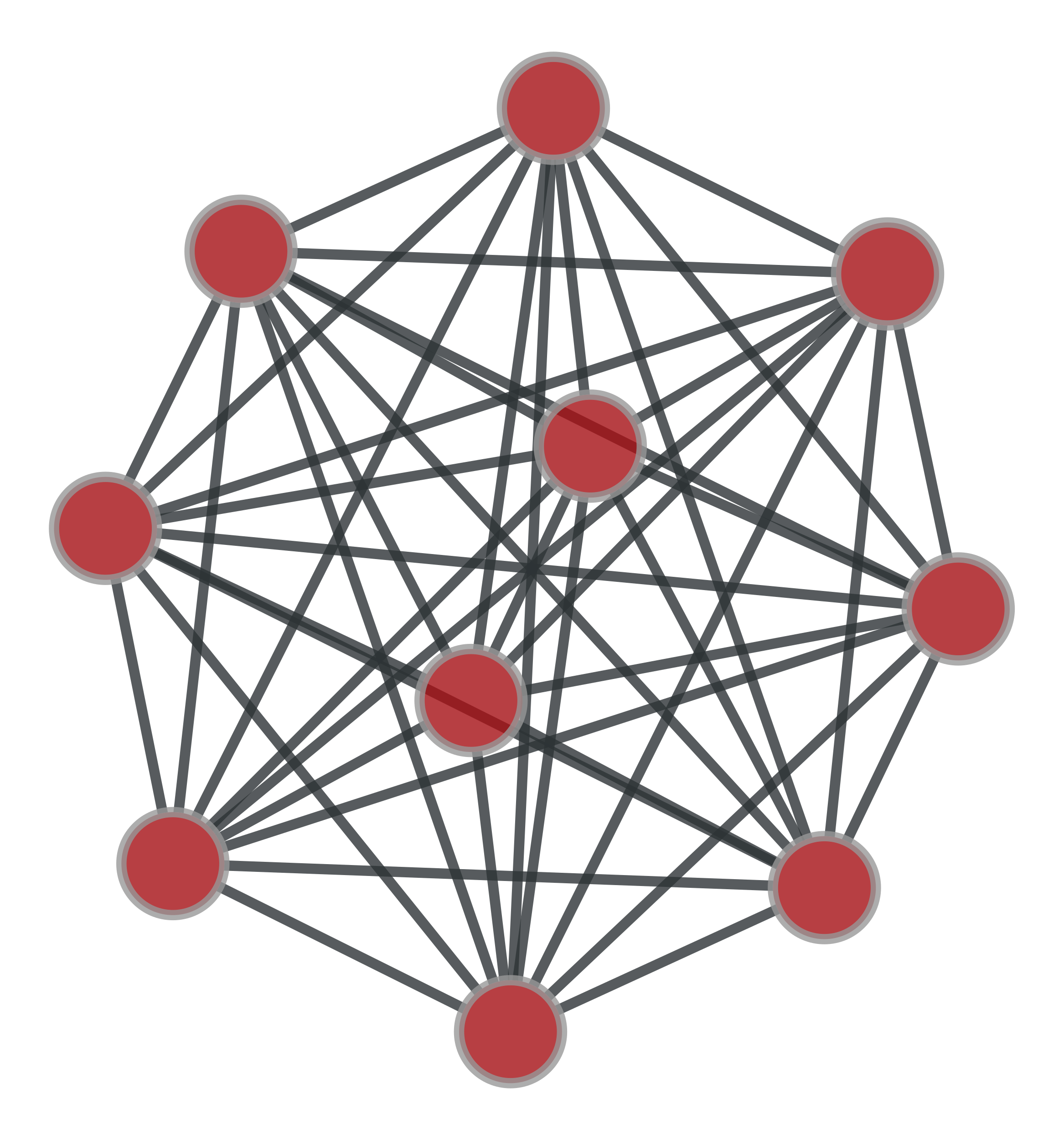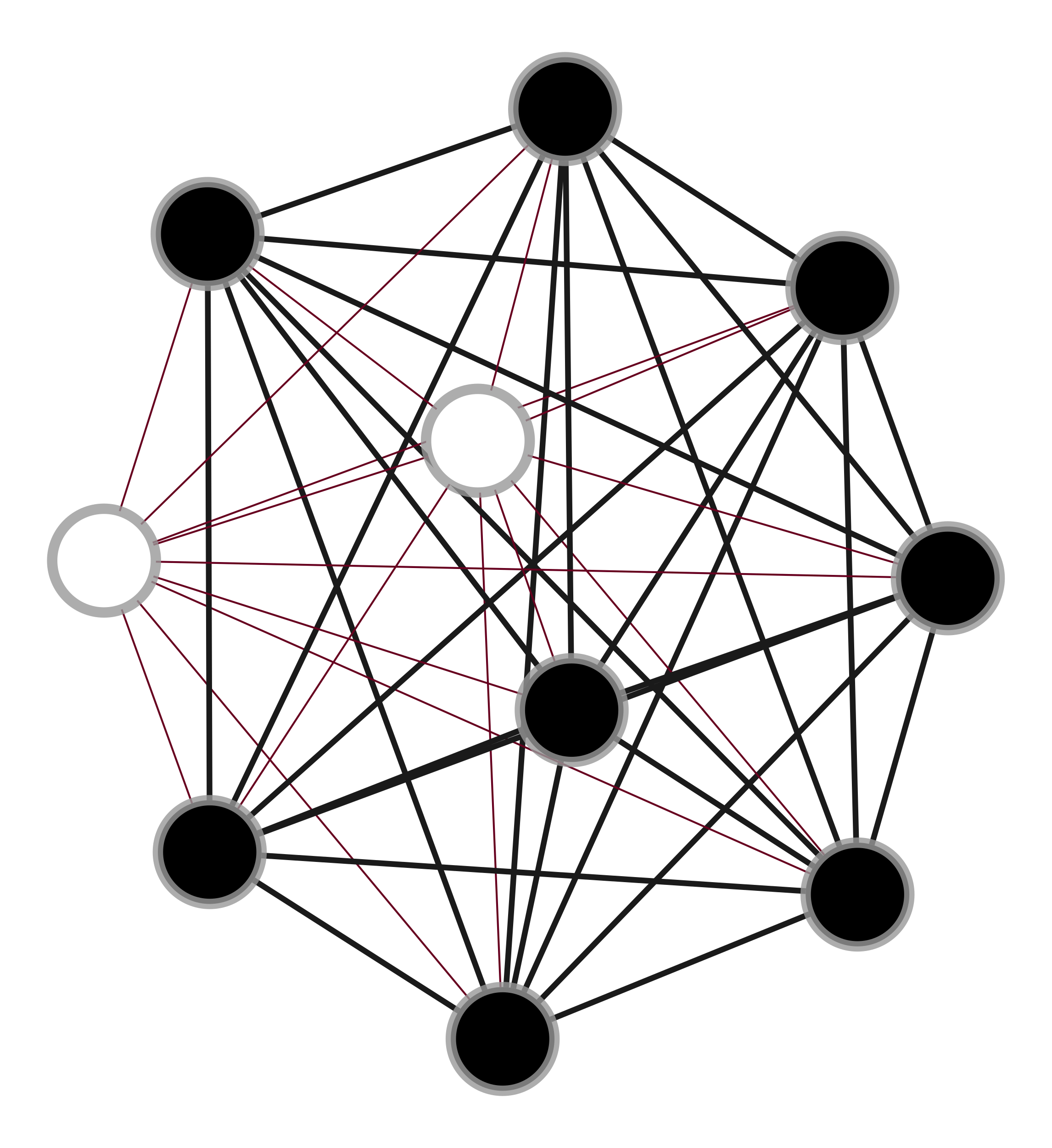Left: Subgraph searched, Right: One isomorphic subgraph found in main graph.

Mark a given subgraph sub on the graph g.

The mapping must be provided by the vmap and emap parameters, which map vertices/edges of sub to indexes of the corresponding vertices/edges in g.

This returns a vertex and an edge property map, with value type ‘bool’, indicating whether or not a vertex/edge in g corresponds to the subgraph sub.

graph_tool.topology.max_cliques(g)[source]

Return an iterator over the maximal cliques of the graph.

Parameters
gGraph

Graph to be used.

Returns
max_cliquesiterator over numpy.ndarray instances

Iterator over lists of vertices corresponding to the maximal cliques.

Notes

This implements the Bron-Kerbosh algorithm [bron_algorithm_1973] [bron-kerbosh-wiki] with pivoting [tomita_worst-case_2006] [cazals_note_2008].

The worst-case complexity of this algorithm is $$O(3^{V/3})$$ for a graph of $$V$$ vertices, but for sparse graphs it is typically much faster.

References

bron_algorithm_1973(1,2)

Coen Bron and Joep Kerbosch, “Algorithm 457: finding all cliques of an undirected graph”, Commun. ACM 16, 9, 575-577 (1973), DOI: 10.1145/362342.362367 [sci-hub, @tor]

tomita_worst-case_2006(1,2)

Etsuji Tomita, Akira Tanaka, and Haruhisa Takahashi. “The worst-case time complexity for generating all maximal cliques and computational experiments.” Theoretical Computer Science 363.1 28-42 (2006), DOI: 10.1016/j.tcs.2006.06.015 [sci-hub, @tor]

cazals_note_2008(1,2)

Frédéric Cazals, and Chinmay Karande, “A note on the problem of reporting maximal cliques.” Theoretical Computer Science 407.1-3 564-568 (2008), DOI: 10.1016/j.tcs.2008.05.010 [sci-hub, @tor]

bron-kerbosh-wiki(1,2)

https://en.wikipedia.org/wiki/Bron%E2%80%93Kerbosch_algorithm

Examples

>>> g = gt.collection.data["polblogs"]
>>> for i, c in enumerate(gt.max_cliques(g)):
...     print(c)
...     if i == 9:
...         break
[513   4]
[  4 720]
[   4  719 1436]
[736   5]
[453   6]
[  7 263  39 753 179 180]
[  7 263  39 179 603]
[  7 263 392  39 753 180 277 223]
[  7 263 392  39 180 277 571 223]
[  7 263  39 603 223]
graph_tool.topology.min_spanning_tree(g, weights=None, root=None, tree_map=None)[source]

Return the minimum spanning tree of a given graph.

Parameters
gGraph

Graph to be used.

weightsEdgePropertyMap (optional, default: None)

The edge weights. If provided, the minimum spanning tree will minimize the edge weights.

rootVertex (optional, default: None)

Root of the minimum spanning tree. If this is provided, Prim’s algorithm is used. Otherwise, Kruskal’s algorithm is used.

tree_mapEdgePropertyMap (optional, default: None)

If provided, the edge tree map will be written in this property map.

Returns
tree_mapEdgePropertyMap

Edge property map with mark the tree edges: 1 for tree edge, 0 otherwise.

Notes

The algorithm runs with $$O(E\log E)$$ complexity, or $$O(E\log V)$$ if root is specified.

References

kruskal-shortest-1956

J. B. Kruskal. “On the shortest spanning subtree of a graph and the traveling salesman problem”, In Proceedings of the American Mathematical Society, volume 7, pages 48-50, 1956. DOI: 10.1090/S0002-9939-1956-0078686-7 [sci-hub, @tor]

prim-shortest-1957

R. Prim. “Shortest connection networks and some generalizations”, Bell System Technical Journal, 36:1389-1401, 1957.

boost-mst

http://www.boost.org/libs/graph/doc/graph_theory_review.html#sec:minimum-spanning-tree

mst-wiki

http://en.wikipedia.org/wiki/Minimum_spanning_tree

Examples

>>> from numpy.random import random
>>> g, pos = gt.triangulation(random((400, 2)) * 10, type="delaunay")
>>> weight = g.new_edge_property("double")
>>> for e in g.edges():
...    weight[e] = linalg.norm(pos[e.target()].a - pos[e.source()].a)
>>> tree = gt.min_spanning_tree(g, weights=weight)
>>> gt.graph_draw(g, pos=pos, output="triang_orig.pdf")
<...>
>>> u = gt.GraphView(g, efilt=tree)
>>> gt.graph_draw(u, pos=pos, output="triang_min_span_tree.pdf")
<...>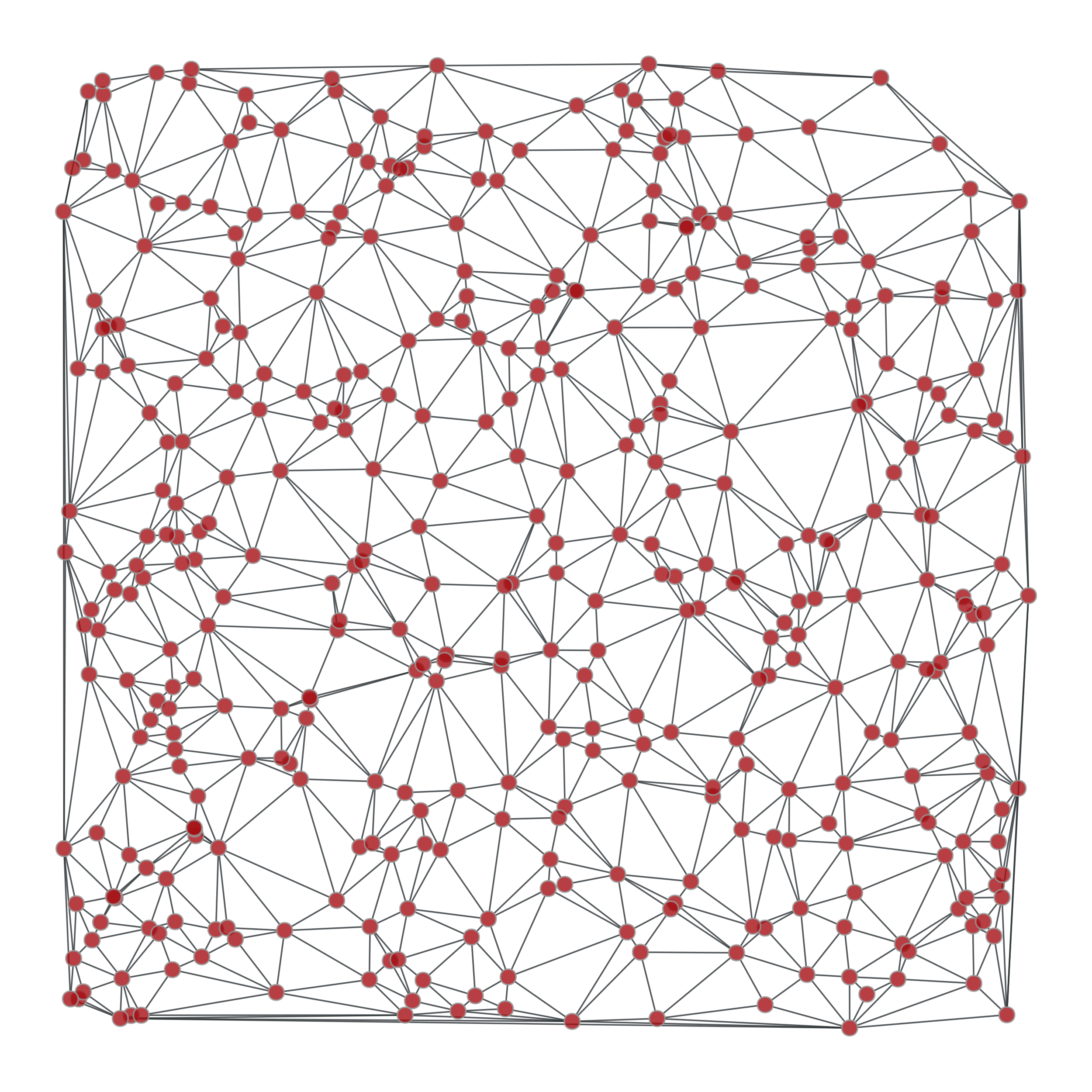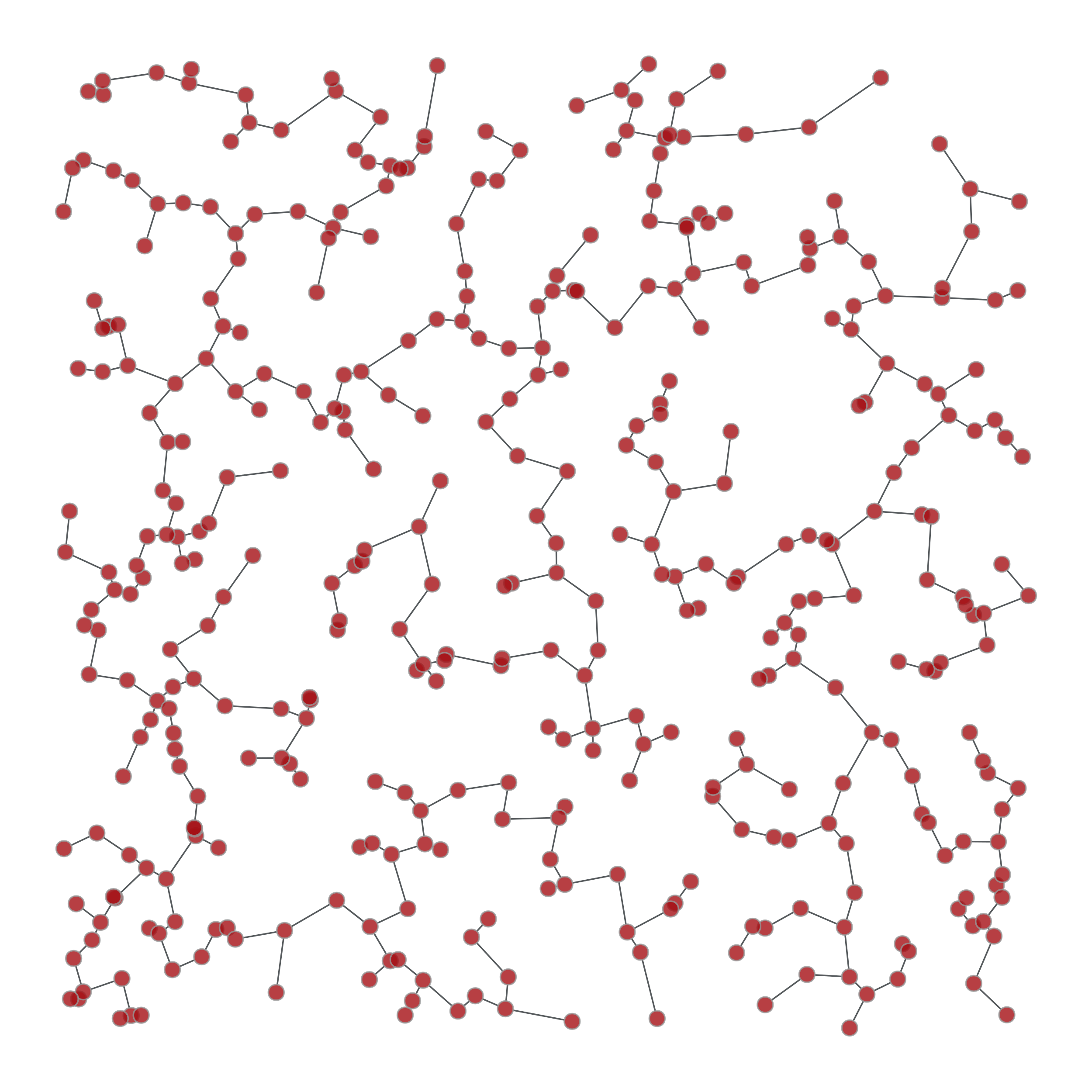Left: Original graph, Right: The minimum spanning tree.

graph_tool.topology.random_spanning_tree(g, weights=None, root=None, tree_map=None)[source]

Return a random spanning tree of a given graph, which can be directed or undirected.

Parameters
gGraph

Graph to be used.

weightsEdgePropertyMap (optional, default: None)

The edge weights. If provided, the probability of a particular spanning tree being selected is the product of its edge weights.

rootVertex (optional, default: None)

Root of the spanning tree. If not provided, it will be selected randomly.

tree_mapEdgePropertyMap (optional, default: None)

If provided, the edge tree map will be written in this property map.

Returns
tree_mapEdgePropertyMap

Edge property map with mark the tree edges: 1 for tree edge, 0 otherwise.

Notes

The running time for this algorithm is $$O(\tau)$$, with $$\tau$$ being the mean hitting time of a random walk on the graph. In the worse case, we have $$\tau \sim O(V^3)$$, with $$V$$ being the number of vertices in the graph. However, in much more typical cases (e.g. sparse random graphs) the running time is simply $$O(V)$$.

References

wilson-generating-1996

David Bruce Wilson, “Generating random spanning trees more quickly than the cover time”, Proceedings of the twenty-eighth annual ACM symposium on Theory of computing, Pages 296-303, ACM New York, 1996, DOI: 10.1145/237814.237880 [sci-hub, @tor]

boost-rst

http://www.boost.org/libs/graph/doc/random_spanning_tree.html

Examples

>>> from numpy.random import random
>>> g, pos = gt.triangulation(random((400, 2)), type="delaunay")
>>> weight = g.new_edge_property("double")
>>> for e in g.edges():
...    weight[e] = linalg.norm(pos[e.target()].a - pos[e.source()].a)
>>> tree = gt.random_spanning_tree(g, weights=weight)
>>> tree2 = gt.random_spanning_tree(g, weights=weight)
>>> gt.graph_draw(g, pos=pos, output="rtriang_orig.pdf")
<...>
>>> u = gt.GraphView(g, efilt=tree)
>>> gt.graph_draw(u, pos=pos, output="triang_random_span_tree.pdf")
<...>
>>> u2 = gt.GraphView(g, efilt=tree2)
>>> gt.graph_draw(u2, pos=pos, output="triang_random_span_tree2.pdf")
<...>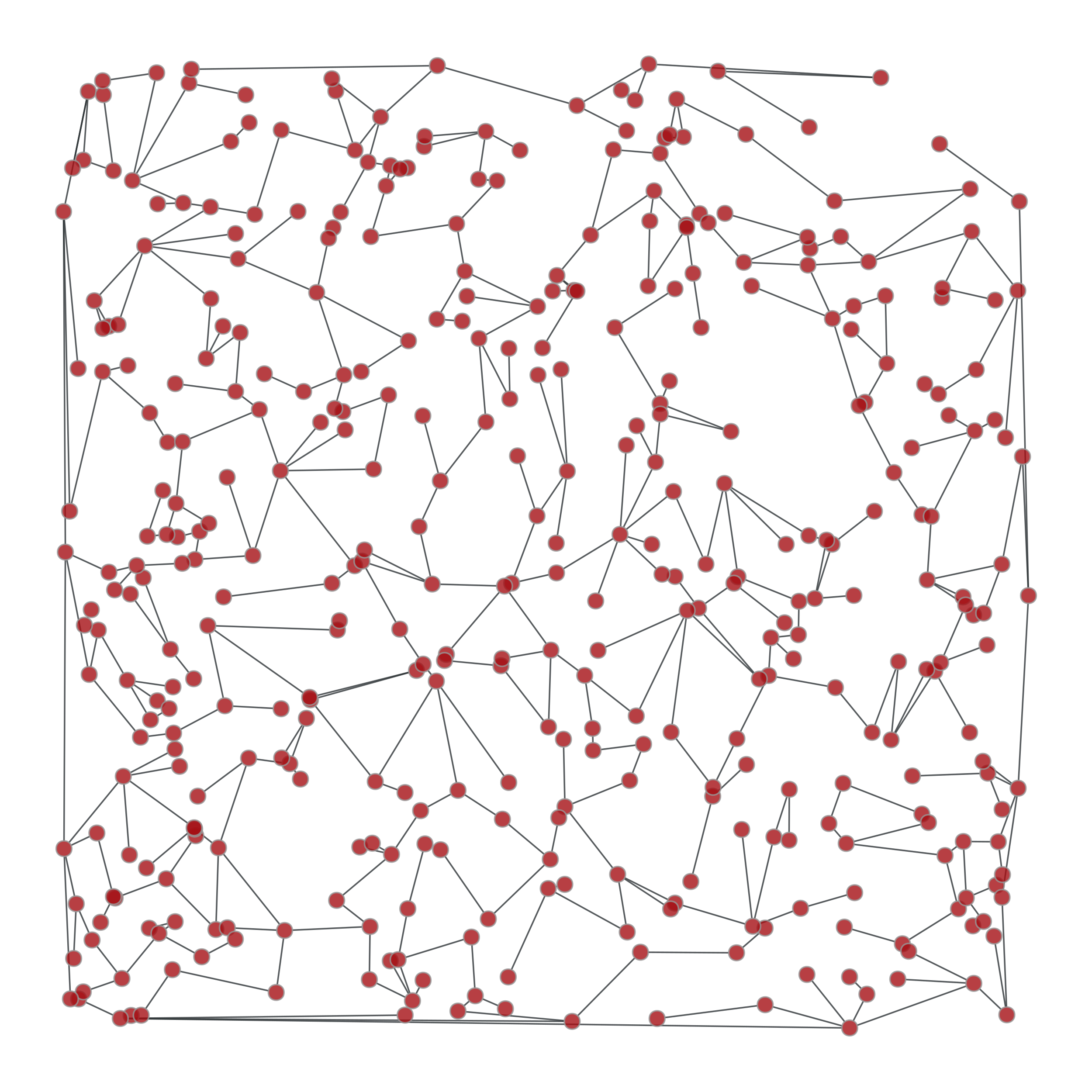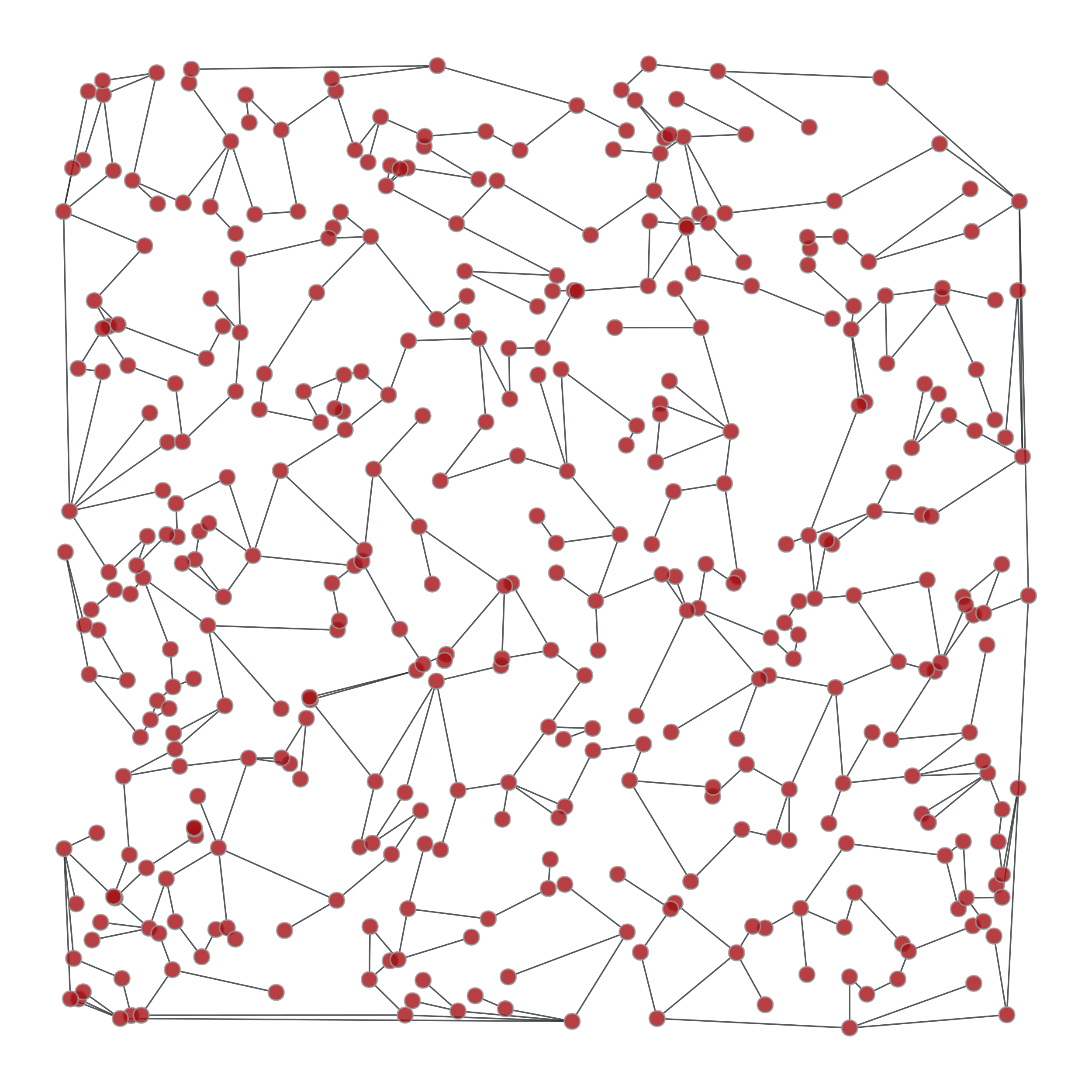Left: Original graph, Middle: A random spanning tree, Right: Another random spanning tree

graph_tool.topology.dominator_tree(g, root, dom_map=None)[source]

Return a vertex property map the dominator vertices for each vertex.

Parameters
gGraph

Graph to be used.

rootVertex

The root vertex.

dom_mapVertexPropertyMap (optional, default: None)

If provided, the dominator map will be written in this property map.

Returns
dom_mapVertexPropertyMap

The dominator map. It contains for each vertex, the index of its dominator vertex.

Notes

A vertex u dominates a vertex v, if every path of directed graph from the entry to v must go through u.

The algorithm runs with $$O((V+E)\log (V+E))$$ complexity.

References

dominator-bgl

http://www.boost.org/libs/graph/doc/lengauer_tarjan_dominator.htm

Examples

>>> g = gt.random_graph(100, lambda: (2, 2))
>>> tree = gt.min_spanning_tree(g)
>>> g.set_edge_filter(tree)
>>> root = [v for v in g.vertices() if v.in_degree() == 0]
>>> dom = gt.dominator_tree(g, root)
>>> print(dom.a)
[ 0  0  0  0  0  0 36  0  0  0  0  0  0  0  0  0  0  0  0  0  0  0  0  0
0  0  0  0  0  0  0  0  0  0  0  0 66  0  0  0  0  0  0  0  0  0  0  0
0  0  0  0  0  0  0  0  0  0  0  0  0  0  0  0  0  0 31  0  0  0  0  0
0  0  0  0  0  0  0  0  0  0  0  0  0  0  0  0  0  0  0  0  0  0  0  0
0  0  0  0]
graph_tool.topology.topological_sort(g)[source]

Return the topological sort of the given graph. It is returned as an array of vertex indexes, in the sort order.

Notes

The topological sort algorithm creates a linear ordering of the vertices such that if edge (u,v) appears in the graph, then u comes before v in the ordering. The graph must be a directed acyclic graph (DAG).

The time complexity is $$O(V + E)$$.

References

topological-boost

http://www.boost.org/libs/graph/doc/topological_sort.html

topological-wiki

http://en.wikipedia.org/wiki/Topological_sorting

Examples

>>> g = gt.random_graph(30, lambda: (3, 3))
>>> tree = gt.min_spanning_tree(g)
>>> g.set_edge_filter(tree)
>>> sort = gt.topological_sort(g)
>>> print(sort)
[28 26 29 25 24 22 21 19 17 16 14 13 10 27  8  5  4 20 12 15  9  2 18  1
0  6 23 11  7  3]
graph_tool.topology.transitive_closure(g)[source]

Return the transitive closure graph of g.

Notes

The transitive closure of a graph G = (V,E) is a graph G* = (V,E*) such that E* contains an edge (u,v) if and only if G contains a path (of at least one edge) from u to v. The transitive_closure() function transforms the input graph g into the transitive closure graph tc.

The time complexity (worst-case) is $$O(VE)$$.

References

transitive-boost

http://www.boost.org/libs/graph/doc/transitive_closure.html

transitive-wiki

http://en.wikipedia.org/wiki/Transitive_closure

Examples

>>> g = gt.random_graph(30, lambda: (3, 3))
>>> tc = gt.transitive_closure(g)
graph_tool.topology.label_components(g, vprop=None, directed=None, attractors=False)[source]

Label the components to which each vertex in the graph belongs. If the graph is directed, it finds the strongly connected components.

A property map with the component labels is returned, together with an histogram of component labels.

Parameters
gGraph

Graph to be used.

vpropVertexPropertyMap (optional, default: None)

Vertex property to store the component labels. If none is supplied, one is created.

directedbool (optional, default: None)

Treat graph as directed or not, independently of its actual directionality.

attractorsbool (optional, default: False)

If True, and the graph is directed, an additional array with Boolean values is returned, specifying if the strongly connected components are attractors or not.

Returns
compVertexPropertyMap

Vertex property map with component labels.

histndarray

Histogram of component labels.

is_attractorndarray

A Boolean array specifying if the strongly connected components are attractors or not. This returned only if attractors == True, and the graph is directed.

Notes

The components are arbitrarily labeled from 0 to N-1, where N is the total number of components.

The algorithm runs in $$O(V + E)$$ time.

Examples

>>> g = gt.random_graph(100, lambda: (poisson(2), poisson(2)))
>>> comp, hist, is_attractor = gt.label_components(g, attractors=True)
>>> print(comp.a)
[ 1 17  4 18 17 19 17 17 17 17 17 15 17 17 20 17 17 17 14 17  0 21 17 17
22 23 16 24 17 17 17 17 25 10 17 17 17  2 27 17  6 13 17 17 17  5 12 17
17 17 26  9 17 17 17  7 17 28 29 17 17  3 30 17 17 17 17 17 17 17 17 17
17 17 17 17 31 17 17 17 17 17 17 17  8 32 17 11 17 17 17 17 33 17 17 17
17 17 17 17]
>>> print(hist)
[ 1  1  1  1  1  1  1  1  1  1  1  1  1  1  1  1  1 67  1  1  1  1  1  1
1  1  1  1  1  1  1  1  1  1]
>>> print(is_attractor)
[ True False  True  True False  True  True False  True False  True  True
True  True False False  True False False False False False False False
True False False False False False  True False False False]
graph_tool.topology.label_largest_component(g, directed=None)[source]

Label the largest component in the graph. If the graph is directed, then the largest strongly connected component is labelled.

A property map with a boolean label is returned.

Parameters
gGraph

Graph to be used.

directedbool (optional, default: None)

Treat graph as directed or not, independently of its actual directionality.

Returns
compVertexPropertyMap

Boolean vertex property map which labels the largest component.

Notes

The algorithm runs in $$O(V + E)$$ time.

Examples

>>> g = gt.random_graph(100, lambda: poisson(1), directed=False)
>>> l = gt.label_largest_component(g)
>>> print(l.a)
[1 0 0 0 0 1 0 0 0 0 0 0 0 0 0 0 0 0 0 0 1 0 1 0 0 0 0 0 0 1 0 0 0 0 0 0 0
0 0 0 0 1 0 0 0 0 0 0 0 0 0 0 0 0 0 0 1 1 0 0 0 0 0 0 0 0 0 0 0 0 0 0 0 0
0 0 0 0 0 1 0 0 0 0 0 0 0 0 1 0 0 0 1 0 0 1 1 1 0 0]
>>> u = gt.GraphView(g, vfilt=l)   # extract the largest component as a graph
>>> print(u.num_vertices())
14
graph_tool.topology.extract_largest_component(g, directed=None, prune=False)[source]

Extract the largest (strong) component in the graph as a GraphView (or Graph if prune==True).

If the graph is directed, then the largest strongly connected component is returned.

Parameters
gGraph

Graph to be used.

directedbool (optional, default: None)

Treat graph as directed or not, independently of its actual directionality.

prunebool (optional, default: False)

If True, a pruned copy of the component is returned. Otherwise a GraphView is returned.

Returns
comp

Largest (strong) component.

Notes

The algorithm runs in $$O(V + E)$$ time.

Examples

>>> g = gt.random_graph(100, lambda: poisson(1), directed=False)
>>> u = gt.extract_largest_component(g)
>>> print(u)
<GraphView object, undirected, with 14 vertices and 13 edges, edges filtered by (<EdgePropertyMap object with value type 'bool', for Graph 0x..., at 0x...>, False), vertices filtered by (<VertexPropertyMap object with value type 'bool', for Graph 0x..., at 0x...>, False) at 0x...>
graph_tool.topology.label_out_component(g, root, label=None)[source]

Label the out-component (or simply the component for undirected graphs) of a root vertex.

Parameters
gGraph

Graph to be used.

rootVertex

The root vertex.

labelVertexPropertyMap (optional, default: None)

If provided, this must be an initialized Boolean vertex property map where the out-component will be labeled.

Returns
labelVertexPropertyMap

Boolean vertex property map which labels the out-component.

Notes

The algorithm runs in $$O(V + E)$$ time.

Examples

>>> g = gt.random_graph(100, lambda: poisson(2.2), directed=False)
>>> l = gt.label_out_component(g, g.vertex(2))
>>> print(l.a)
[1 1 1 1 1 0 0 1 1 0 0 0 1 1 1 1 1 1 1 1 1 1 1 1 1 1 1 1 1 1 1 0 0 1 1 1 1
1 1 0 1 1 1 1 1 1 1 1 0 1 1 1 0 1 0 1 1 0 0 1 1 1 1 1 1 0 0 1 0 1 1 1 1 1
1 1 1 0 1 1 1 1 1 1 1 1 1 1 1 1 1 0 1 1 1 0 1 1 1 0]

The in-component can be obtained by reversing the graph.

>>> l = gt.label_out_component(gt.GraphView(g, reversed=True, directed=True),
...                            g.vertex(1))
>>> print(l.a)
[0 1 0 0 0 0 0 0 0 0 0 0 0 0 0 0 0 0 0 0 0 0 0 0 0 0 0 0 0 0 0 0 0 0 0 0 0
0 0 0 0 0 0 0 0 0 0 0 0 0 0 0 0 0 0 0 0 0 0 0 0 0 0 0 0 0 0 0 0 0 0 0 0 0
0 0 0 0 0 0 0 0 0 0 0 0 0 0 0 0 0 0 0 0 0 0 0 0 0 0]
graph_tool.topology.label_biconnected_components(g, eprop=None, vprop=None)[source]

Label the edges of biconnected components, and the vertices which are articulation points.

An edge property map with the component labels is returned, together a boolean vertex map marking the articulation points, and an histogram of component labels.

Parameters
gGraph

Graph to be used.

epropEdgePropertyMap (optional, default: None)

Edge property to label the biconnected components.

vpropVertexPropertyMap (optional, default: None)

Vertex property to mark the articulation points. If none is supplied, one is created.

Returns
bicompEdgePropertyMap

Edge property map with the biconnected component labels.

articulationVertexPropertyMap

Boolean vertex property map which has value 1 for each vertex which is an articulation point, and zero otherwise.

ncint

Number of biconnected components.

Notes

A connected graph is biconnected if the removal of any single vertex (and all edges incident on that vertex) can not disconnect the graph. More generally, the biconnected components of a graph are the maximal subsets of vertices such that the removal of a vertex from a particular component will not disconnect the component. Unlike connected components, vertices may belong to multiple biconnected components: those vertices that belong to more than one biconnected component are called “articulation points” or, equivalently, “cut vertices”. Articulation points are vertices whose removal would increase the number of connected components in the graph. Thus, a graph without articulation points is biconnected. Vertices can be present in multiple biconnected components, but each edge can only be contained in a single biconnected component.

The algorithm runs in $$O(V + E)$$ time.

Examples

>>> g = gt.random_graph(100, lambda: poisson(2), directed=False)
>>> comp, art, hist = gt.label_biconnected_components(g)
>>> print(comp.a)
[45 45 45 45 45 21 45 27 14 45 45 45 45 45 17 45 45 45 45 45 45 45 19 20
45 45 44 45 42 37 45 45 45 45 45 45 38 24 28 45 45 45 45 29 45  6 34 45
2 45 45  9 45 36 33 30 45 45 45 11 23 45 47 45 45 45 45 25  1 48 12 39
18  7 31 40 45 45 13  5  4 45 16 45  8 45  0 45 26 22 10 46  3 50 41 49
43 32 45 35 15]
>>> print(art.a)
[1 0 0 1 0 1 0 0 1 1 0 0 0 1 0 1 1 1 0 0 1 0 0 0 0 0 0 0 0 1 1 0 0 1 0 0 0
1 0 0 0 1 1 0 0 1 1 0 0 1 1 0 0 1 1 1 0 1 0 0 0 0 1 1 0 1 1 0 0 0 0 0 0 1
0 0 1 0 0 1 0 1 1 0 0 1 1 0 0 1 0 0 0 0 0 0 1 1 0 0]
>>> print(hist)
[ 1  1  1  1  1  1  1  1  1  1  1  1  1  1  1  1  1  1  1  1  1  1  1  1
1  1  1  1  1  1  1  1  1  1  1  1  1  1  1  1  1  1  1  1  1 51  1  1
1  1  1]
graph_tool.topology.vertex_percolation(g, vertices, second=False)[source]

Compute the size of the largest or second-largest component as vertices are (virtually) removed from the graph.

Parameters
gGraph

Graph to be used.

verticesnumpy.ndarray or iterable of ints

List of vertices in reversed order of removal.

secondbool (optional, default: False)

If True, the size of the second-largest component will be computed.

Returns
sizenumpy.ndarray

Size of the largest (or second-largest) component prior to removal of each vertex.

compVertexPropertyMap

Vertex property map with component labels.

Notes

The algorithm runs in $$O(V + E)$$ time.

References

newman-ziff

M. E. J. Newman, R. M. Ziff, “A fast Monte Carlo algorithm for site or bond percolation”, Phys. Rev. E 64, 016706 (2001) DOI: 10.1103/PhysRevE.64.016706 [sci-hub, @tor], arXiv: cond-mat/0101295

Examples

>>> g = gt.random_graph(10000, lambda: geometric(1./4) + 1, directed=False)
>>> vertices = sorted([v for v in g.vertices()], key=lambda v: v.out_degree())
>>> sizes, comp = gt.vertex_percolation(g, vertices)
>>> numpy.random.shuffle(vertices)
>>> sizes2, comp = gt.vertex_percolation(g, vertices)
>>> figure()
<...>
>>> plot(sizes, label="Targeted")
[...]
>>> plot(sizes2, label="Random")
[...]
>>> xlabel("Vertices remaining")
Text(...)
>>> ylabel("Size of largest component")
Text(...)
>>> legend(loc="upper left")
<...>
>>> savefig("vertex-percolation.svg")Targeted and random vertex percolation of a random graph with an exponential degree distribution.

graph_tool.topology.edge_percolation(g, edges, second=False)[source]

Compute the size of the largest or second-largest component as edges are (virtually) removed from the graph.

Parameters
gGraph

Graph to be used.

edgesnumpy.ndarray or iterable of pairs of ints

List of edges in reversed order of removal. If the type is numpy.ndarray, it should have a shape (E, 2), where E is the number of edges, such that edges[i,0] and edges[i,1] are the both endpoints of edge i.

secondbool (optional, default: False)

If True, the size of the second-largest component will be computed.

Returns
sizenumpy.ndarray

Size of the largest (or second-largest) component prior to removal of each edge.

compVertexPropertyMap

Vertex property map with component labels.

Notes

The algorithm runs in $$O(E)$$ time.

References

newman-ziff

M. E. J. Newman, R. M. Ziff, “A fast Monte Carlo algorithm for site or bond percolation”, Phys. Rev. E 64, 016706 (2001) DOI: 10.1103/PhysRevE.64.016706 [sci-hub, @tor], arXiv: cond-mat/0101295

Examples

>>> g = gt.random_graph(10000, lambda: geometric(1./4) + 1, directed=False)
>>> edges = sorted([(e.source(), e.target()) for e in g.edges()],
...                key=lambda e: e.out_degree() * e.out_degree())
>>> sizes, comp = gt.edge_percolation(g, edges)
>>> numpy.random.shuffle(edges)
>>> sizes2, comp = gt.edge_percolation(g, edges)
>>> figure()
<...>
>>> plot(sizes, label="Targeted")
[...]
>>> plot(sizes2, label="Random")
[...]
>>> xlabel("Edges remaining")
Text(...)
>>> ylabel("Size of largest component")
Text(...)
>>> legend(loc="lower right")
<...>
>>> savefig("edge-percolation.svg")Targeted and random edge percolation of a random graph with an exponential degree distribution.

graph_tool.topology.kcore_decomposition(g, vprop=None)[source]

Perform a k-core decomposition of the given graph.

Parameters
gGraph

Graph to be used.

vpropVertexPropertyMap (optional, default: None)

Vertex property to store the decomposition. If None is supplied, one is created.

Returns
kvalVertexPropertyMap

Vertex property map with the k-core decomposition, i.e. a given vertex v belongs to the kval[v]-core.

Notes

The k-core is a maximal set of vertices such that its induced subgraph only contains vertices with degree larger than or equal to k.

For directed graphs, the degree is assumed to be the total (in + out) degree.

The algorithm accepts graphs with parallel edges and self loops, in which case these edges contribute to the degree in the usual fashion.

This algorithm is described in [batagelj-algorithm] and runs in $$O(V + E)$$ time.

References

k-core

http://en.wikipedia.org/wiki/Degeneracy_%28graph_theory%29

batagelj-algorithm(1,2)

Vladimir Batagelj, Matjaž Zaveršnik, “Fast algorithms for determining (generalized) core groups in social networks”, Advances in Data Analysis and Classification Volume 5, Issue 2, pp 129-145 (2011), DOI: 10.1007/s11634-010-0079-y [sci-hub, @tor], arXiv: cs/0310049

Examples

>>> g = gt.collection.data["netscience"]
>>> g = gt.GraphView(g, vfilt=gt.label_largest_component(g))
>>> kcore = gt.kcore_decomposition(g)
>>> gt.graph_draw(g, pos=g.vp["pos"], vertex_fill_color=kcore, vertex_text=kcore, output="netsci-kcore.pdf")
<...>K-core decomposition of a network of network scientists.

graph_tool.topology.shortest_distance(g, source=None, target=None, weights=None, negative_weights=False, max_dist=None, directed=None, dense=False, dist_map=None, pred_map=False, return_reached=False, dag=False)[source]

Calculate the distance from a source to a target vertex, or to of all vertices from a given source, or the all pairs shortest paths, if the source is not specified.

Parameters
gGraph

Graph to be used.

sourceVertex (optional, default: None)

Source vertex of the search. If unspecified, the all pairs shortest distances are computed.

targetVertex or iterable of such objects (optional, default: None)

Target vertex (or vertices) of the search. If unspecified, the distance to all vertices from the source will be computed.

weightsEdgePropertyMap (optional, default: None)

The edge weights. If provided, the shortest path will correspond to the minimal sum of weights.

negative_weightsbool (optional, default: False)

If True, this will trigger the use of the Bellman-Ford algorithm. Ignored if source is None.

max_distscalar value (optional, default: None)

If specified, this limits the maximum distance of the vertices searched. This parameter has no effect if source is None, or if negative_weights=True.

directedbool (optional, default:None)

Treat graph as directed or not, independently of its actual directionality.

densebool (optional, default: False)

If True, and source is None, the Floyd-Warshall algorithm is used, otherwise the Johnson algorithm is used. If source is not None, this option has no effect.

dist_mapVertexPropertyMap (optional, default: None)

Vertex property to store the distances. If none is supplied, one is created.

Warning

If this parameter is supplied, the user is responsible for initializing it to infinity. This can be done as:

>>> dist_map = g.new_vp("double", numpy.inf)

or

>>> dist_map = g.new_vp("int32_t", numpy.iinfo(numpy.int32).max)

depending on the distance type.

pred_mapbool or VertexPropertyMap (optional, default: False)

If True, a vertex property map with the predecessors is returned. If a VertexPropertyMap is passed, it must be of value int64_t and it will be used to store the predecessors. Ignored if source is None.

Warning

If a property map is supplied, the user is responsible for initializing to the identity map. This can be done as:

>>> pred_map = g.vertex_index.copy()
return_reachedbool (optional, default: False)

If True, return an array of visited vertices.

dagbool (optional, default:False)

If True, assume that the graph is a Directed Acyclic Graph (DAG), which will be faster if weights are given, in which case they are also allowed to contain negative values (irrespective of the parameter negative_weights).

Returns
dist_map

Vertex property map with the distances from source. If source is None, it will have a vector value type, with the distances to every vertex. If target is an iterable, instead of VertexPropertyMap, this will be of type numpy.ndarray, and contain only the distances to those specific targets.

pred_mapVertexPropertyMap (optional, if pred_map == True)

Vertex property map with the predecessors in the search tree.

pred_mapnumpy.ndarray (optional, if return_reached == True)

Array containing vertices visited during the search.

Notes

If a source is given, the distances are calculated with a breadth-first search (BFS) or Dijkstra’s algorithm [dijkstra], if weights are given. If negative_weights == True, the Bellman-Ford algorithm is used [bellman-ford], which accepts negative weights, as long as there are no negative loops. If source is not given, the distances are calculated with Johnson’s algorithm [johnson-apsp]. If dense=True, the Floyd-Warshall algorithm [floyd-warshall-apsp] is used instead.

If there is no path between two vertices, the computed distance will correspond to the maximum value allowed by the value type of dist_map, or inf in case of floating point types.

If source is specified, the algorithm runs in $$O(V + E)$$ time, or $$O(V \log V)$$ if weights are given (if dag == True this improves to $$O(V+E)$$). If negative_weights == True, the complexity is $$O(VE)$$. If source is not specified, it runs in $$O(VE\log V)$$ time, or $$O(V^3)$$ if dense == True.

References

bfs

Edward Moore, “The shortest path through a maze”, International Symposium on the Theory of Switching (1959), Harvard University Press.

bfs-boost

dijkstra(1,2)

E. Dijkstra, “A note on two problems in connexion with graphs.” Numerische Mathematik, 1:269-271, 1959.

dijkstra-boost

http://www.boost.org/libs/graph/doc/dijkstra_shortest_paths.html

johnson-apsp(1,2)

http://www.boost.org/libs/graph/doc/johnson_all_pairs_shortest.html

floyd-warshall-apsp(1,2)

http://www.boost.org/libs/graph/doc/floyd_warshall_shortest.html

bellman-ford(1,2)

http://www.boost.org/libs/graph/doc/bellman_ford_shortest.html

Examples

>>> from numpy.random import poisson
>>> g = gt.random_graph(100, lambda: (poisson(3), poisson(3)))
>>> dist = gt.shortest_distance(g, source=g.vertex(0))
>>> print(dist.a)
[         0          5          6          3 2147483647          6
6          6          4          4          5          5
4          3          7          6          4          5
6          5          4          6          4          3
6          6          4          5          5          3
4          4          5          4          4          6
3 2147483647          3          5 2147483647          2
5          9          6          6          5          4
7          5          4          2          4          6
4          1          4          6          5          3
4          5          4          5          4          5
7          8          3          5 2147483647 2147483647
3          6          5          5          5          5
5          3          7          2          7          5
3          5          5          5          7          4
5          6          4          5          4          5
4          6          5          4]
>>>
>>> dist = gt.shortest_distance(g)
>>> print(dist[g.vertex(0)].a)
[         0          5          6          3 2147483647          6
6          6          4          4          5          5
4          3          7          6          4          5
6          5          4          6          4          3
6          6          4          5          5          3
4          4          5          4          4          6
3 2147483647          3          5 2147483647          2
5          9          6          6          5          4
7          5          4          2          4          6
4          1          4          6          5          3
4          5          4          5          4          5
7          8          3          5 2147483647 2147483647
3          6          5          5          5          5
5          3          7          2          7          5
3          5          5          5          7          4
5          6          4          5          4          5
4          6          5          4]
>>> dist = gt.shortest_distance(g, source=g.vertex(0), target=g.vertex(2))
>>> print(dist)
6
>>> dist = gt.shortest_distance(g, source=g.vertex(0), target=[g.vertex(2), g.vertex(6)])
>>> print(dist)
[6 6]
graph_tool.topology.shortest_path(g, source, target, weights=None, negative_weights=False, pred_map=None, dag=False)[source]

Return the shortest path from source to target.

Parameters
gGraph

Graph to be used.

sourceVertex

Source vertex of the search.

targetVertex

Target vertex of the search.

weightsEdgePropertyMap (optional, default: None)

The edge weights.

negative_weightsbool (optional, default: False)

If True, this will trigger the use of the Bellman-Ford algorithm.

pred_mapVertexPropertyMap (optional, default: None)

Vertex property map with the predecessors in the search tree. If this is provided, the shortest paths are not computed, and are obtained directly from this map.

dagbool (optional, default:False)

If True, assume that the graph is a Directed Acyclic Graph (DAG), which will be faster if weights are given, in which case they are also allowed to contain negative values (irrespective of the parameter negative_weights).

Returns
vertex_listlist of Vertex

List of vertices from source to target in the shortest path.

edge_listlist of Edge

List of edges from source to target in the shortest path.

Notes

The paths are computed with a breadth-first search (BFS) or Dijkstra’s algorithm [dijkstra], if weights are given. If negative_weights == True, the Bellman-Ford algorithm is used [bellman-ford], which accepts negative weights, as long as there are no negative loops.

The algorithm runs in $$O(V + E)$$ time, or $$O(V \log V)$$ if weights are given (if dag == True this improves to $$O(V+E)$$).

References

bfs

Edward Moore, “The shortest path through a maze”, International Symposium on the Theory of Switching (1959), Harvard University Press

bfs-boost

dijkstra(1,2)

E. Dijkstra, “A note on two problems in connexion with graphs.” Numerische Mathematik, 1:269-271, 1959.

dijkstra-boost

http://www.boost.org/libs/graph/doc/dijkstra_shortest_paths.html

bellman-ford(1,2)

http://www.boost.org/libs/graph/doc/bellman_ford_shortest.html

Examples

>>> from numpy.random import poisson
>>> g = gt.random_graph(300, lambda: (poisson(4), poisson(4)))
>>> vlist, elist = gt.shortest_path(g, g.vertex(10), g.vertex(11))
>>> print([str(v) for v in vlist])
['10', '272', '225', '245', '11']
>>> print([str(e) for e in elist])
['(10, 272)', '(272, 225)', '(225, 245)', '(245, 11)']
graph_tool.topology.all_predecessors(g, dist_map, pred_map, weights=None, epsilon=1e-08)[source]
Return a property map with all possible predecessors in the search tree

determined by dist_map and pred_map.

Parameters
gGraph

Graph to be used.

dist_mapVertexPropertyMap

Vertex property map with the distances from source to all other vertices.

pred_mapVertexPropertyMap (optional, default: None)

Vertex property map with the predecessors in the search tree.

weightsEdgePropertyMap (optional, default: None)

The edge weights.

epsilonfloat (optional, default: 1e-8)

Maximum relative difference between distances to be considered “equal”, in case floating-point weights are used.

Returns
all_preds_mapVertexPropertyMap

Vector-valued vertex property map with all possible predecessors in the search tree.

graph_tool.topology.all_shortest_paths(g, source, target, weights=None, negative_weights=False, dist_map=None, pred_map=None, all_preds_map=None, epsilon=1e-08, dag=False)[source]

Return an iterator over all shortest paths from source to target.

Parameters
gGraph

Graph to be used.

sourceVertex

Source vertex of the search.

targetVertex

Target vertex of the search.

weightsEdgePropertyMap (optional, default: None)

The edge weights.

negative_weightsbool (optional, default: False)

If True, this will trigger the use of the Bellman-Ford algorithm.

dist_mapVertexPropertyMap (optional, default: None)

Vertex property map with the distances from source to all other vertices.

pred_mapVertexPropertyMap (optional, default: None)

Vertex property map with the predecessors in the search tree. If this is provided, the shortest paths are not computed, and are obtained directly from this map.

all_preds_mapVertexPropertyMap (optional, default: None)

Vector-valued vertex property map with all possible predecessors in the search tree. If this is provided, the shortest paths are obtained directly from this map.

epsilonfloat (optional, default: 1e-8)

Maximum relative difference between distances to be considered “equal”, in case floating-point weights are used.

dagbool (optional, default:False)

If True, assume that the graph is a Directed Acyclic Graph (DAG), which will be faster if weights are given, in which case they are also allowed to contain negative values (irrespective of the parameter negative_weights).

Returns
path_iteratoriterator over a sequence of integers

Iterator over sequences of vertices from source to target in the shortest path.

Notes

The paths are computed with a breadth-first search (BFS) or Dijkstra’s algorithm [dijkstra], if weights are given. If negative_weights == True, the Bellman-Ford algorithm is used [bellman-ford], which accepts negative weights, as long as there are no negative loops.

If both dist_map and pred_map are provided, the search is not actually performed.

References

bfs

Edward Moore, “The shortest path through a maze”, International Symposium on the Theory of Switching (1959), Harvard University Press

bfs-boost

dijkstra(1,2)

E. Dijkstra, “A note on two problems in connexion with graphs.” Numerische Mathematik, 1:269-271, 1959.

dijkstra-boost

http://www.boost.org/libs/graph/doc/dijkstra_shortest_paths.html

bellman-ford(1,2)

http://www.boost.org/libs/graph/doc/bellman_ford_shortest.html

Examples

>>> g = gt.collection.data["pgp-strong-2009"]
>>> for path in gt.all_shortest_paths(g, 92, 45):
...     print(path)
[  92  107 2176 7027   26   21   45]
[  92  107 2176 7033   26   21   45]
[  92   82   94 5877 5879   34   45]
[  92   89   94 5877 5879   34   45]
graph_tool.topology.all_paths(g, source, target, cutoff=None, edges=False)[source]

Return an iterator over all paths from source to target.

Parameters
gGraph

Graph to be used.

sourceVertex

Source vertex of the search.

targetVertex

Target vertex of the search.

cutoffint (optional, default: None)

Maximum path length.

edgesbool (optional, default: False)

If True, the returned iterator is over edge descriptors.

Returns
path_iteratoriterator over a sequence of integers (or Edge)

Iterator over sequences of vertices from source to target in the path. If edges == True, the iterator is over sequences of edge descriptors (Edge).

Notes

The algorithm uses a depth-first search to find all the paths.

The total number of paths between any two vertices can be quite large, possibly scaling as $$O(V!)$$.

Examples

>>> g = gt.collection.data["football"]
>>> for path in gt.all_paths(g, 13, 2, cutoff=2):
...     print(path)
[13  2]
[13 15  2]
[13 60  2]
[13 64  2]
[ 13 100   2]
[ 13 106   2]
graph_tool.topology.all_circuits(g, unique=False)[source]

Return an iterator over all the cycles in a directed graph.

Parameters
gGraph

A directed graph to be used.

uniquebool (optional, default: None)

If True, parallel edges and self-loops will be ignored.

Returns
cycle_iteratoriterator over a sequence of integers

Iterator over sequences of vertices that form a circuit.

Notes

This algorithm [hawick-enumerating-2008] runs in worse time $$O[(V + E)(C + 1)]$$, where $$C$$ is the number of circuits.

References

hawick-enumerating-2008(1,2)

K.A. Hawick and H.A. James, “Enumerating Circuits and Loops in Graphs with Self-Arcs and Multiple-Arcs.”, In Proceedings of FCS. 2008, 14-20, http://cssg.massey.ac.nz/cstn/013/cstn-013.html

hawick-bgl

http://www.boost.org/doc/libs/graph/doc/hawick_circuits.html

Examples

>>> g = gt.random_graph(10, lambda: (1, 1))
>>> for c in gt.all_circuits(g):
...     print(c)
[0 1 6 3 8 4 9]
[2 5 7]
graph_tool.topology.pseudo_diameter(g, source=None, weights=None)[source]

Compute the pseudo-diameter of the graph.

Parameters
gGraph

Graph to be used.

sourceVertex (optional, default: None)

Source vertex of the search. If not supplied, the first vertex in the graph will be chosen.

weightsEdgePropertyMap (optional, default: None)

The edge weights.

Returns
pseudo_diameterint

The pseudo-diameter of the graph.

end_pointspair of Vertex

The two vertices which correspond to the pseudo-diameter found.

Notes

The pseudo-diameter is an approximate graph diameter. It is obtained by starting from a vertex source, and finds a vertex target that is farthest away from source. This process is repeated by treating target as the new starting vertex, and ends when the graph distance no longer increases. A vertex from the last level set that has the smallest degree is chosen as the final starting vertex u, and a traversal is done to see if the graph distance can be increased. This graph distance is taken to be the pseudo-diameter.

The paths are computed with a breadth-first search (BFS) or Dijkstra’s algorithm [dijkstra], if weights are given.

The algorithm runs in $$O(V + E)$$ time, or $$O(V \log V)$$ if weights are given.

References

pseudo-diameter

http://en.wikipedia.org/wiki/Distance_%28graph_theory%29

dijkstra(1,2)

E. Dijkstra, “A note on two problems in connexion with graphs.” Numerische Mathematik, 1:269-271, 1959.

Examples

>>> from numpy.random import poisson
>>> g = gt.random_graph(300, lambda: (poisson(3), poisson(3)))
>>> dist, ends = gt.pseudo_diameter(g)
>>> print(dist)
9.0
>>> print(int(ends), int(ends))
294 259
graph_tool.topology.is_bipartite(g, partition=False, find_odd_cycle=False)[source]

Test if the graph is bipartite.

Parameters
gGraph

Graph to be used.

partitionbool (optional, default: False)

If True, return the two partitions in case the graph is bipartite.

find_odd_cyclebool (optional, default: False)

If True, return an odd cycle if the graph is not bipartite.

Returns
is_bipartitebool

Whether or not the graph is bipartite.

partitionVertexPropertyMap (only if partition=True)

A vertex property map with the graph partitioning (or None) if the graph is not bipartite.

odd_cyclelist of vertices (only if find_odd_cycle=True)

A list of vertices corresponding to an odd cycle, or None if none is found.

Notes

An undirected graph is bipartite if one can partition its set of vertices into two sets, such that all edges go from one set to the other.

This algorithm runs in $$O(V + E)$$ time.

References

boost-bipartite

http://www.boost.org/libs/graph/doc/is_bipartite.html

Examples

>>> g = gt.lattice([10, 10])
>>> is_bi, part = gt.is_bipartite(g, partition=True)
>>> print(is_bi)
True
>>> gt.graph_draw(g, vertex_fill_color=part, output_size=(300, 300), output="bipartite.pdf")
<...>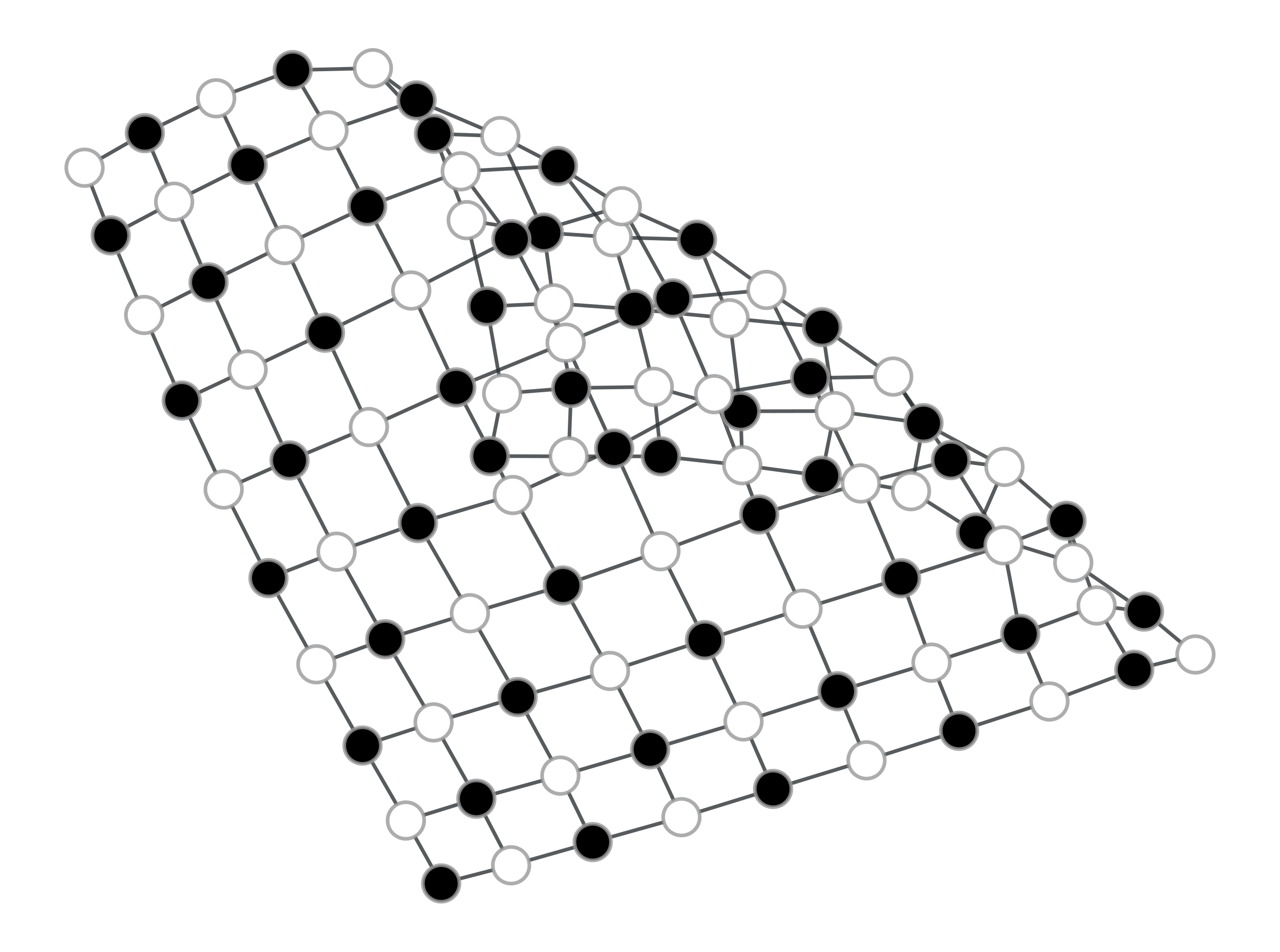Bipartition of a 2D lattice.

graph_tool.topology.is_planar(g, embedding=False, kuratowski=False)[source]

Test if the graph is planar.

Parameters
gGraph

Graph to be used.

embeddingbool (optional, default: False)

If true, return a mapping from vertices to the clockwise order of out-edges in the planar embedding.

kuratowskibool (optional, default: False)

If true, the minimal set of edges that form the obstructing Kuratowski subgraph will be returned as a property map, if the graph is not planar.

Returns
is_planarbool

Whether or not the graph is planar.

embeddingVertexPropertyMap (only if embedding=True)

A vertex property map with the out-edges indexes in clockwise order in the planar embedding,

kuratowskiEdgePropertyMap (only if kuratowski=True)

An edge property map with the minimal set of edges that form the obstructing Kuratowski subgraph (if the value of kuratowski[e] is 1, the edge belongs to the set)

Notes

A graph is planar if it can be drawn in two-dimensional space without any of its edges crossing. This algorithm performs the Boyer-Myrvold planarity testing [boyer-myrvold]. See [boost-planarity] for more details.

This algorithm runs in $$O(V)$$ time.

References

boyer-myrvold(1,2)

John M. Boyer and Wendy J. Myrvold, “On the Cutting Edge: Simplified O(n) Planarity by Edge Addition” Journal of Graph Algorithms and Applications, 8(2): 241-273, 2004. http://www.emis.ams.org/journals/JGAA/accepted/2004/BoyerMyrvold2004.8.3.pdf

boost-planarity(1,2)

http://www.boost.org/libs/graph/doc/boyer_myrvold.html

Examples

>>> from numpy.random import random
>>> g = gt.triangulation(random((100,2)))
>>> p, embed_order = gt.is_planar(g, embedding=True)
>>> print(p)
True
>>> print(list(embed_order[g.vertex(0)]))
[0, 2, 3, 4, 5, 6, 7, 8, 9, 10, 11, 12, 13, 14, 15, 16, 17, 18, 19, 20, 21, 22, 23, 24, 1]
>>> g = gt.random_graph(100, lambda: 4, directed=False)
>>> p, kur = gt.is_planar(g, kuratowski=True)
>>> print(p)
False
>>> g.set_edge_filter(kur, True)
>>> gt.graph_draw(g, output_size=(300, 300), output="kuratowski.pdf")
<...>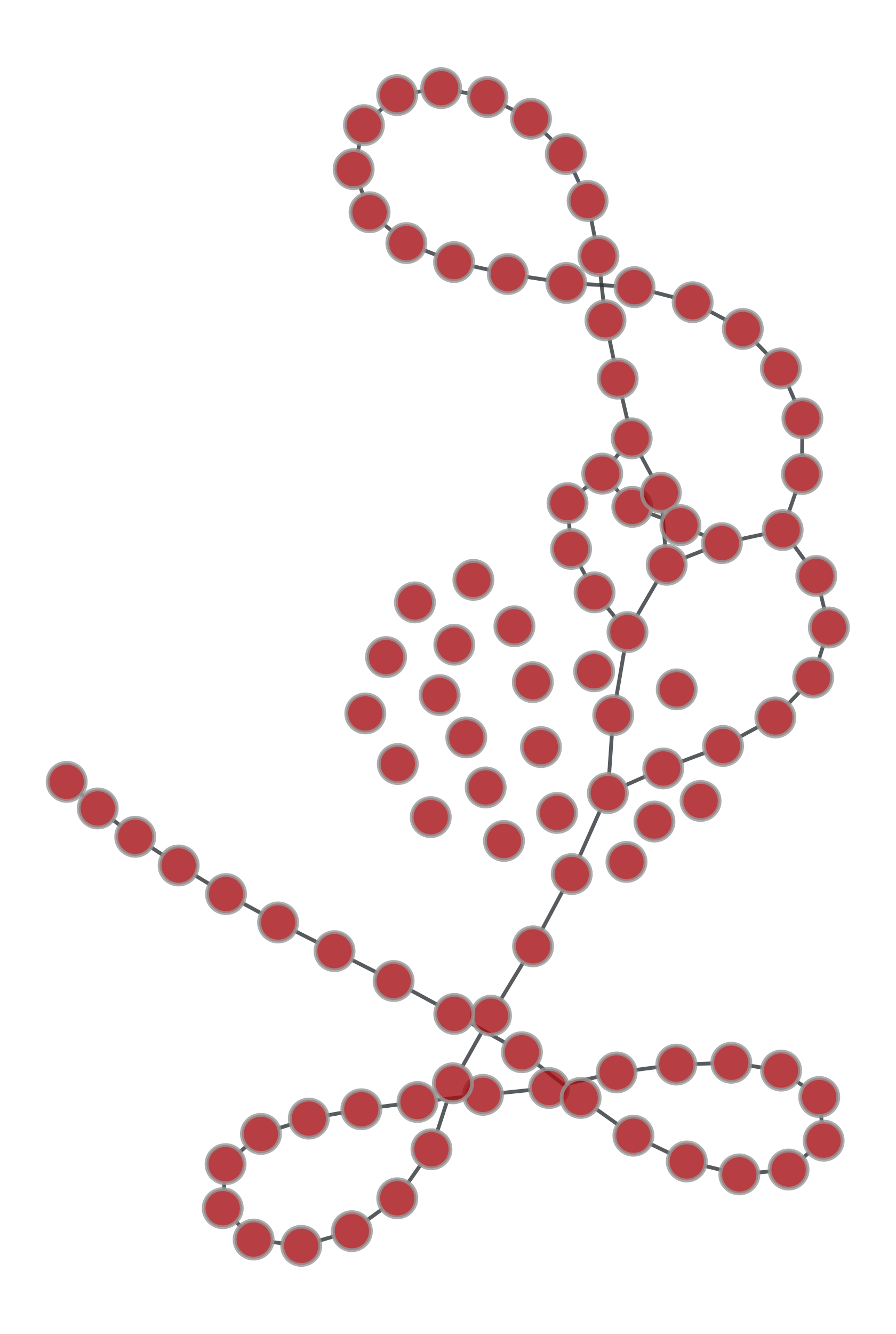Obstructing Kuratowski subgraph of a random graph.

graph_tool.topology.make_maximal_planar(g)[source]

Add edges to the graph to make it maximally planar.

Parameters
gGraph

Graph to be used. It must be a biconnected planar graph with at least 3 vertices.

Notes

A graph is maximal planar if no additional edges can be added to it without creating a non-planar graph. By Euler’s formula, a maximal planar graph with V > 2 vertices always has 3V - 6 edges and 2V - 4 faces.

The input graph to make_maximal_planar() must be a biconnected planar graph with at least 3 vertices.

This algorithm runs in $$O(V + E)$$ time.

References

boost-planarity

http://www.boost.org/libs/graph/doc/make_maximal_planar.html

Examples

>>> g = gt.lattice([10, 10])
>>> gt.make_maximal_planar(g)
>>> gt.is_planar(g)
True
>>> print(g.num_vertices(), g.num_edges())
100 294
>>> pos = gt.planar_layout(g)
>>> gt.graph_draw(g, pos, output_size=(300, 300), output="maximal_planar.pdf")
<...>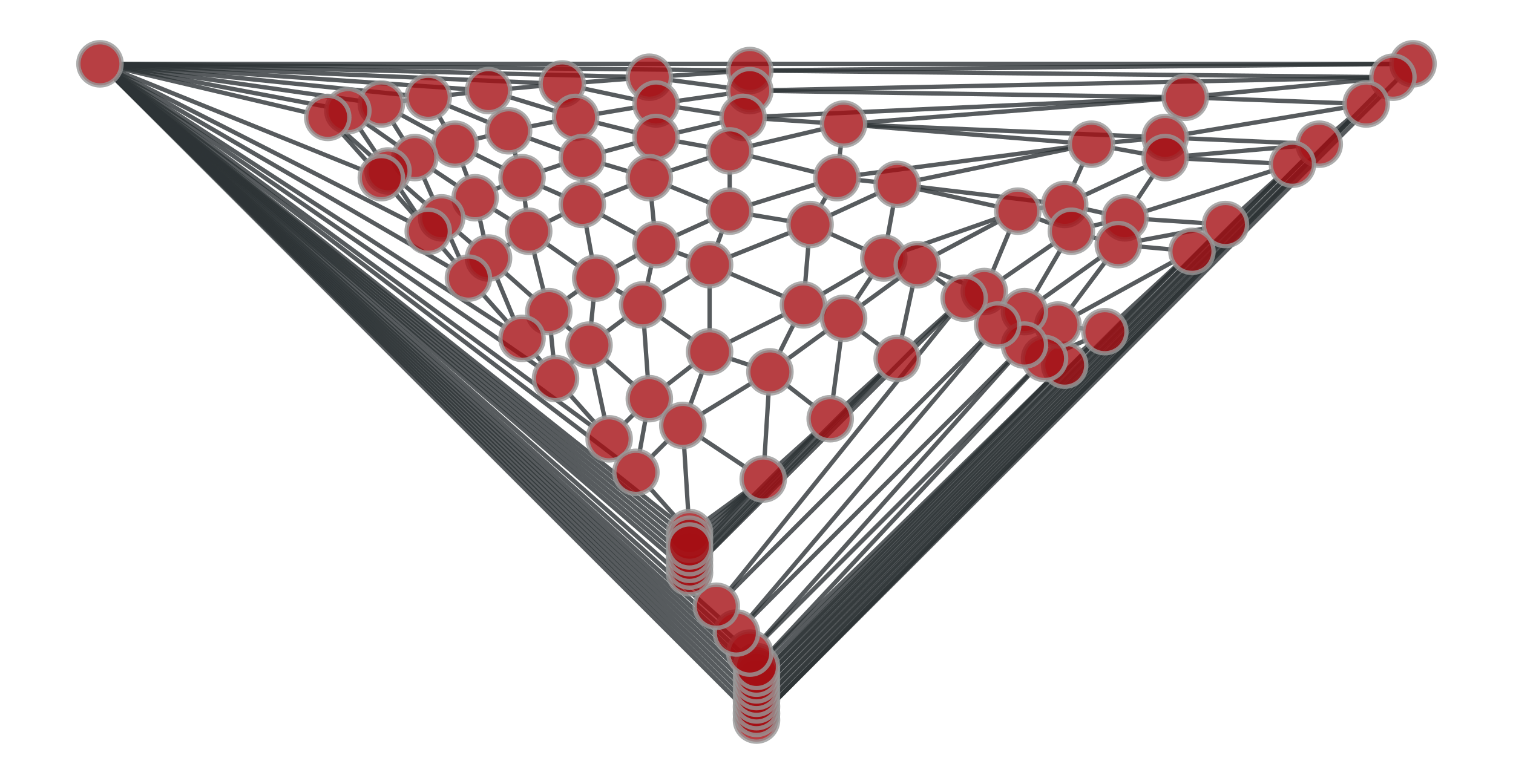A maximally planar graph.

graph_tool.topology.is_DAG(g)[source]

Return True if the graph is a directed acyclic graph (DAG).

Notes

The time complexity is $$O(V + E)$$.

References

DAG-wiki

http://en.wikipedia.org/wiki/Directed_acyclic_graph

Examples

>>> g = gt.random_graph(30, lambda: (3, 3))
>>> print(gt.is_DAG(g))
False
>>> tree = gt.min_spanning_tree(g)
>>> g.set_edge_filter(tree)
>>> print(gt.is_DAG(g))
True
graph_tool.topology.max_cardinality_matching(g, heuristic=False, weight=None, minimize=True, match=None)[source]

Find a maximum cardinality matching in the graph.

Parameters
gGraph

Graph to be used.

heuristicbool (optional, default: False)

If true, a random heuristic will be used, which runs in linear time.

weightEdgePropertyMap (optional, default: None)

If provided, the matching will minimize the edge weights (or maximize if minimize == False). This option has no effect if heuristic == False.

minimizebool (optional, default: True)

If True, the matching will minimize the weights, otherwise they will be maximized. This option has no effect if heuristic == False.

matchEdgePropertyMap (optional, default: None)

Edge property map where the matching will be specified.

Returns
matchEdgePropertyMap

Boolean edge property map where the matching is specified.

Notes

A matching is a subset of the edges of a graph such that no two edges share a common vertex. A maximum cardinality matching has maximum size over all matchings in the graph.

If the parameter weight is provided, as well as heuristic == True a matching with maximum cardinality and maximum (or minimum) weight is returned.

If heuristic == True the algorithm does not necessarily return the maximum matching, instead the focus is to run on linear time.

This algorithm runs in time $$O(EV\times\alpha(E,V))$$, where $$\alpha(m,n)$$ is a slow growing function that is at most 4 for any feasible input. If heuristic == True, the algorithm runs in time $$O(V + E)$$.

For a more detailed description, see [boost-max-matching].

References

boost-max-matching(1,2)

http://www.boost.org/libs/graph/doc/maximum_matching.html

matching-heuristic

B. Hendrickson and R. Leland. “A Multilevel Algorithm for Partitioning Graphs.” In S. Karin, editor, Proc. Supercomputing ’95, San Diego. ACM Press, New York, 1995, DOI: 10.1145/224170.224228 [sci-hub, @tor]

Examples

>>> g = gt.GraphView(gt.price_network(300), directed=False)
>>> res = gt.max_cardinality_matching(g)
>>> print(res)
True
>>> w = res.copy("double")
>>> w.a = 2 * w.a + 2
>>> gt.graph_draw(g, edge_color=res, edge_pen_width=w, vertex_fill_color="grey",
...               output="max_card_match.pdf")
<...>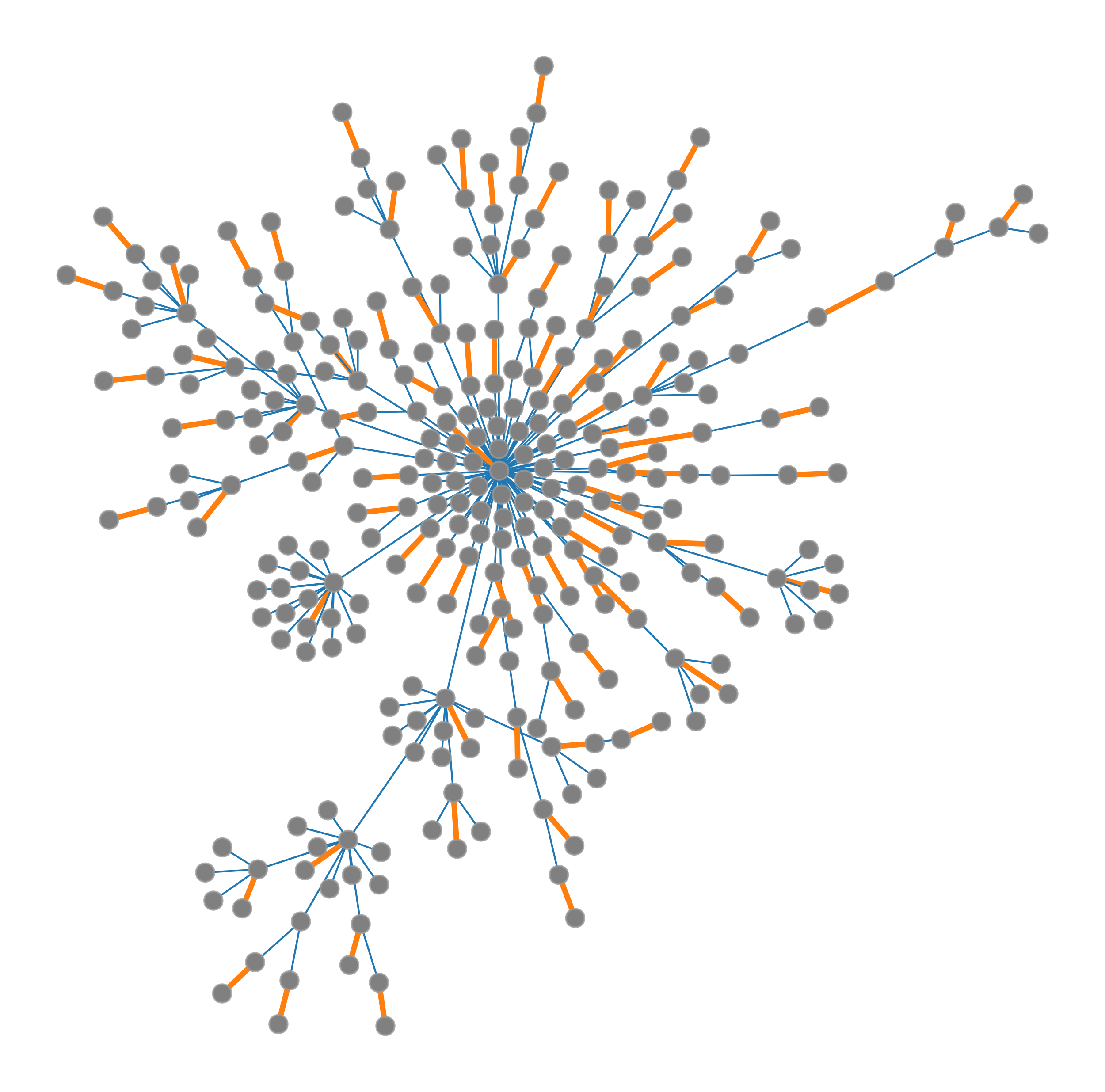Edges belonging to the matching are in yellow.

graph_tool.topology.max_independent_vertex_set(g, high_deg=False, mivs=None)[source]

Find a maximal independent vertex set in the graph.

Parameters
gGraph

Graph to be used.

high_degbool (optional, default: False)

If True, vertices with high degree will be included first in the set, otherwise they will be included last.

mivsVertexPropertyMap (optional, default: None)

Vertex property map where the vertex set will be specified.

Returns
mivsVertexPropertyMap

Boolean vertex property map where the set is specified.

Notes

A maximal independent vertex set is an independent set such that adding any other vertex to the set forces the set to contain an edge between two vertices of the set.

This implements the algorithm described in [mivs-luby], which runs in time $$O(V + E)$$.

References

mivs-wikipedia

http://en.wikipedia.org/wiki/Independent_set_%28graph_theory%29

mivs-luby(1,2)

Luby, M., “A simple parallel algorithm for the maximal independent set problem”, Proc. 17th Symposium on Theory of Computing, Association for Computing Machinery, pp. 1-10, (1985) DOI: 10.1145/22145.22146 [sci-hub, @tor].

Examples

>>> g = gt.GraphView(gt.price_network(300), directed=False)
>>> res = gt.max_independent_vertex_set(g)
>>> gt.graph_draw(g, vertex_fill_color=res, output="mivs.pdf")
<...>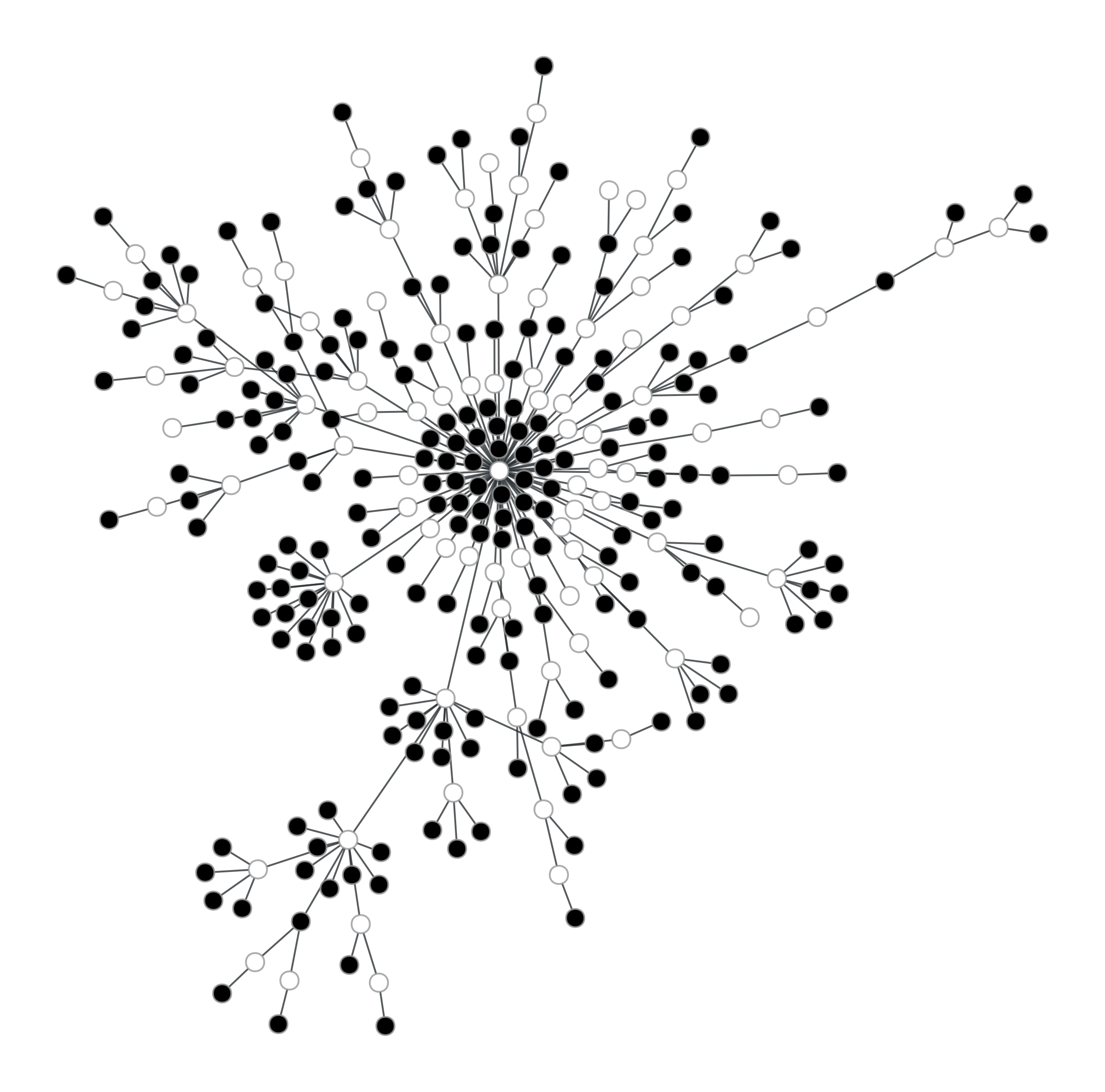Vertices belonging to the set are in yellow.

graph_tool.topology.edge_reciprocity(g)[source]

Calculate the edge reciprocity of the graph.

Parameters
gGraph

Graph to be used edges.

Returns
reciprocityfloat

The reciprocity value.

Notes

The edge [reciprocity] is defined as $$E^\leftrightarrow/E$$, where $$E^\leftrightarrow$$ and $$E$$ are the number of bidirectional and all edges in the graph, respectively.

The algorithm runs with complexity $$O(E + V)$$.

References

reciprocity(1,2)

S. Wasserman and K. Faust, “Social Network Analysis”. (Cambridge University Press, Cambridge, 1994)

lopez-reciprocity-2007

Gorka Zamora-López, Vinko Zlatić, Changsong Zhou, Hrvoje Štefančić, and Jürgen Kurths “Reciprocity of networks with degree correlations and arbitrary degree sequences”, Phys. Rev. E 77, 016106 (2008) DOI: 10.1103/PhysRevE.77.016106 [sci-hub, @tor], arXiv: 0706.3372

Examples

>>> g = gt.Graph()
<...>
<...>
>>> gt.edge_reciprocity(g)
0.0
<...>
>>> gt.edge_reciprocity(g)
1.0
>>> g = gt.collection.data["pgp-strong-2009"]
>>> gt.edge_reciprocity(g)
0.692196963163...
graph_tool.topology.tsp_tour(g, src, weight=None)[source]

Return a traveling salesman tour of the graph, which is guaranteed to be twice as long as the optimal tour in the worst case.

Parameters
gGraph

Graph to be used. The graph must be undirected.

srcVertex

The source (and target) of the tour.

weightEdgePropertyMap (optional, default: None)

Edge weights.

Returns
tournumpy.ndarray

List of vertex indexes corresponding to the tour.

Notes

The algorithm runs with $$O(E\log V)$$ complexity.

References

tsp-bgl

http://www.boost.org/libs/graph/doc/metric_tsp_approx.html

tsp

http://en.wikipedia.org/wiki/Travelling_salesman_problem

Examples

>>> g = gt.lattice([10, 10])
>>> tour = gt.tsp_tour(g, g.vertex(0))
>>> print(tour)
[ 0  1  2 11 12 21 22 31 32 41 42 51 52 61 62 71 72 81 82 83 73 63 53 43
33 23 13  3  4  5  6  7  8  9 19 29 39 49 59 69 79 89 14 24 34 44 54 64
74 84 91 92 93 94 95 85 75 65 55 45 35 25 15 16 17 18 27 28 37 38 47 48
57 58 67 68 77 78 87 88 97 98 99 26 36 46 56 66 76 86 96 10 20 30 40 50
60 70 80 90  0]
graph_tool.topology.sequential_vertex_coloring(g, order=None, color=None)[source]

Returns a vertex coloring of the graph.

Parameters
gGraph

Graph to be used.

orderVertexPropertyMap (optional, default: None)

Order with which the vertices will be colored.

colorVertexPropertyMap (optional, default: None)

Integer-valued vertex property map to store the colors.

Returns
colorVertexPropertyMap

Integer-valued vertex property map with the vertex colors.

Notes

The time complexity is $$O(V(d+k))$$, where $$V$$ is the number of vertices, $$d$$ is the maximum degree of the vertices in the graph, and $$k$$ is the number of colors used.

References

sgc-bgl

http://www.boost.org/libs/graph/doc/sequential_vertex_coloring.html

graph-coloring

http://en.wikipedia.org/wiki/Graph_coloring

Examples

>>> g = gt.lattice([10, 10])
>>> colors = gt.sequential_vertex_coloring(g)
>>> print(colors.a)
[0 1 0 1 0 1 0 1 0 1 1 0 1 0 1 0 1 0 1 0 0 1 0 1 0 1 0 1 0 1 1 0 1 0 1 0 1
0 1 0 0 1 0 1 0 1 0 1 0 1 1 0 1 0 1 0 1 0 1 0 0 1 0 1 0 1 0 1 0 1 1 0 1 0
1 0 1 0 1 0 0 1 0 1 0 1 0 1 0 1 1 0 1 0 1 0 1 0 1 0]